• 想当年，我学车的时候也是糊里糊涂啊，练了天都没练好，30公分到底是什么鬼？而且不仅是科目二要30公分，科目三也要用到30公分！！！所以说，学会判断30公分，是考车成功的第要诀啊！ 首先，从车外看，30公分是...
想当年，我学车的时候也是糊里糊涂啊，练了一天都没练好，30公分到底是什么鬼？而且不仅是科目二要30公分，科目三也要用到30公分！！！所以说，学会判断30公分，是考车成功的第一要诀啊！

首先，从车外看，30公分是这样的。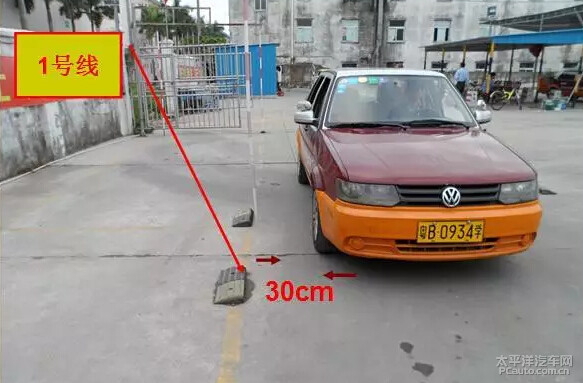然后，带你们看一下车内的30公分是咋样的（通过右后视镜来看）。嗯？。。你们问我，什么是30公分？其实就是30厘米啦！

牢记上面这个图，看黄线和车身的距离，30公分，差不多就是这样啦。

先说一下，科目二考试有什么地方需要用到30公分！

1、侧方停车

侧方停车一开始倒库就必须准确找到车身距离黄线的30公分的长度。

侧方停车的30公分和上面这个图有些相似，就是直接进去的时候必须距离黄线30公分，要不然倒库的时候会导致角度过小或者过大，后续就会出现很多问题的，所以一开始要把30公分看清楚。

倒车入库如何把握车身与库角30厘米?这个问题困扰了许多想学修库的朋友，开始总把握不准这个30公分，在后视镜里是缩小的虚像，在后视镜面上可以用两个指头或一只笔放在镜面上可以盖死它，但是它随着车的位置不同会有变化，如果我们不按具体情况来区别那就不好把握，所以把握不准的主要原因是没有根据倒库早晚的程度和车的位置来感知这个30公分。
下面是我的做法：以右倒库为例。首先在库口距右库角大约30厘米的地方做一个标志，(如上图)可以用树叶，黄泥巴，粉笔----(不过一旦掌握了，30公分在脑海里有影像了，必须撤下标志不能再依赖它)然后要分四种情况去感知这个30公分，(以右倒库为例)第一种情况倒库标准点30公分，右倒库在标准点右打死，看右后视镜，发现在标准点这种情况下，车身慢慢靠近右库角，在某一时刻右车身会开始盖住标志点，但始终没有把这个标志全盖住，至少还可以看到一点点标志，也就是车离库最近距离大约是30厘米，这个时候的30公分就是倒库标准点30公分，最后进库时后轮外沿恰好压着这个标志点进去，完美入库。为了更好的把握，我们可以用手机对着右后视镜把这种情况拍下来。
第二种情况倒库早一点30公分
右倒库比标准点提前一个手指距离右打死，看右后视镜，发现在早一点这种情况下，车身慢慢靠近右库角，在某一时刻右车身会开始盖住标志点，这个时刻的30公分就是倒库早一点30公分，同时发现这个时刻的到来比上一种情况来的早一点，若不采取行动，车继续倒，那么车身会把这个标志全盖住，并且还要往库角靠近，这时我们要果断的把方向往左打，打多少不要管，只要还可以看到一点点标志就停打，也就是车离库最近距离不允许少于30厘米，为了更好的把握，我们可以用手机对着右后视镜把这种情况也拍下来。
第三种情况倒库较早点30公分
右倒库比标准点提前两个或三个手指距离右打死，看右后视镜，发现在较早点这种情况下，车身靠近右库角速度加快，在某一时刻右车身会开始盖住标志点，这个时刻的30公分就是倒库较早点30公分，同时发现这个时刻的到来比上一种情况来的还要早一点，若不采取行动，车身很快会把这个标志全盖住，并且还要快速往库角靠近，这时我们要果断的把方向往左打，打多少不要管，只要还可以看到一点点标志就停打，也就是车离库最近距离不允许少于30厘米，为了更好的把握，我们可以用手机对着右后视镜把这种情况也拍下来。
第四种情况倒库超早点30公分，右倒库比标准点提前一个巴掌以上距离右打死，看右后视镜，发现在超早点这种情况下，车身靠近右库角速度更快，在某一时刻右车身会开始盖住标志点，这个时刻的30公分就是倒库超早点30公分，同时发现这个时刻的到来比上一种情况来的还要早一点，若不采取行动，车身很快会把这个标志全盖住，还会去压库角，这时我们要果断的把方向往左打，打540度也就是回直车身，必须保证能看到一点点标志，也就是车离库最近距离不允许少于30厘米，为了更好的把握，我们可以请人用手机对着右后视镜把这种情况也拍下来。也可以自己在车身开始盖标志的时候把车停下来，用手机在驾驶位置对准后视镜把这些各个不同情况的30公分拍照下来。
最后通过多次观看，进行比较发现在不同情况30公分给人的感觉是不同的，标准点30公分感觉最宽，超早点30公分感觉最少，其实都是30公分宽，为什么有区别，这是因为车离库口的距离不同，点位打的越早30公分的极限来的越早，车这时离库越远，同时修方向的时机也要提前。知道上面四种情况。我们必须多次练习去感知这四种情况，把这四种情况的30公分影像嵌入自己的脑海，同时借助拍下的视频图片加深印象，把拍下的图片放在电脑和手机做壁纸，每天去加深印象，那么我们在倒库时看后视镜车和库角距离的变化立即可以按四种情况对号入座，点位打早了，什么时候开始修方向，到底修多少也就不难了。

展开全文• 各种比例尺标准分经差、纬差表比例尺1:100万1:50万1:25万1:10万1:5万1:2.5万1:11:5千经差6º3º1º30′30′15′7′30″3′45″1′52.5″纬差4º2º1
 各种比例尺标准分幅图经差、纬差表

比例尺
1:100万
1:50万
1:25万
1:10万
1:5万
1:2.5万
1:1万
1:5千
经差
6º
3º
1º30′
30′
15′
7′30″
3′45″
1′52.5″
纬差
4º
2º
1º
20′
10′
5′
2′30″
1′15″


展开全文• 1. 这本书对Python的知识点的描述很详细，而且排版看的很舒服. 2. 几个例题：假装自己从零开始学，将一些有代表性、有意思的例题抽取出来. 3. 还有自己对部分课后复习题，全部课后上机实践题的解题思路
（还在更新中…） 这篇博客花费了我的大量时间和精力，从创作到维护；若认可本篇博客，希望给一个点赞、收藏
并且，遇到了什么问题，请在评论区留言，我会及时回复的

这本书对Python的知识点的描述很详细，而且排版看的很舒服

几个例题： 假装自己从零开始学，将一些有代表性、有意思的例题抽取出来部分复习题： 遇到有意思的复习题，我会拿出来，并且进行分析上机实践： 全部上机实践题的解题思路

文章目录
第一章 Python概述几个例题
第二章 Python语言基础选择题：1、3、7、8思考题：9上机实践：2~6案例研究：使用Pillow库处理图像文件
第三章 程序流程控制几个例题选择题：1、2、3填空题：6思考题：3~6上机实践：2~14案例研究：使用嵌套循环实现图像处理算法
第四章 常用内置数据类型几个例题选择题：11填空题：4、7、8、9、10、13、21思考题：5上机实践：2~14案例研究：科学计算和数据分析
第五章 序列数据类型几个例题选择题：4、5、7、11、12填空题：1、5、6、12、13、14思考题：2、3、5上机实践：2~6案例研究：猜单词游戏
第六章 输入和输出几个例题填空题：1、2例题及上机实践：2~5案例研究：21点扑克牌游戏
第七章 错误和异常处理几个例题案例研究：使用调试器调试Python程序
第八章 函数和函数式编程一些知识点总结和几个例题选择题：1~5思考题：4~11上机实践：2~5案例研究：井字棋游戏
第九章 面向对象的程序设计第十章 模块和客户端第十一章 算法与数据结构基础第十二章 图形用户界面案例研究：简易图形用户界面计算器
第十三章 图形绘制图形绘制模块：tkinter图形绘制模块：turtle
第十四章 数值日期和时间处理第十五章 字符串和文本处理第十六章 文件和数据交换第十七章 数据访问第十八章 网络编程和通信第十九章 并行计算：进程、线程和协程第二十章 系统管理

第一章 Python概述

几个例题
一：Python3.7.4下载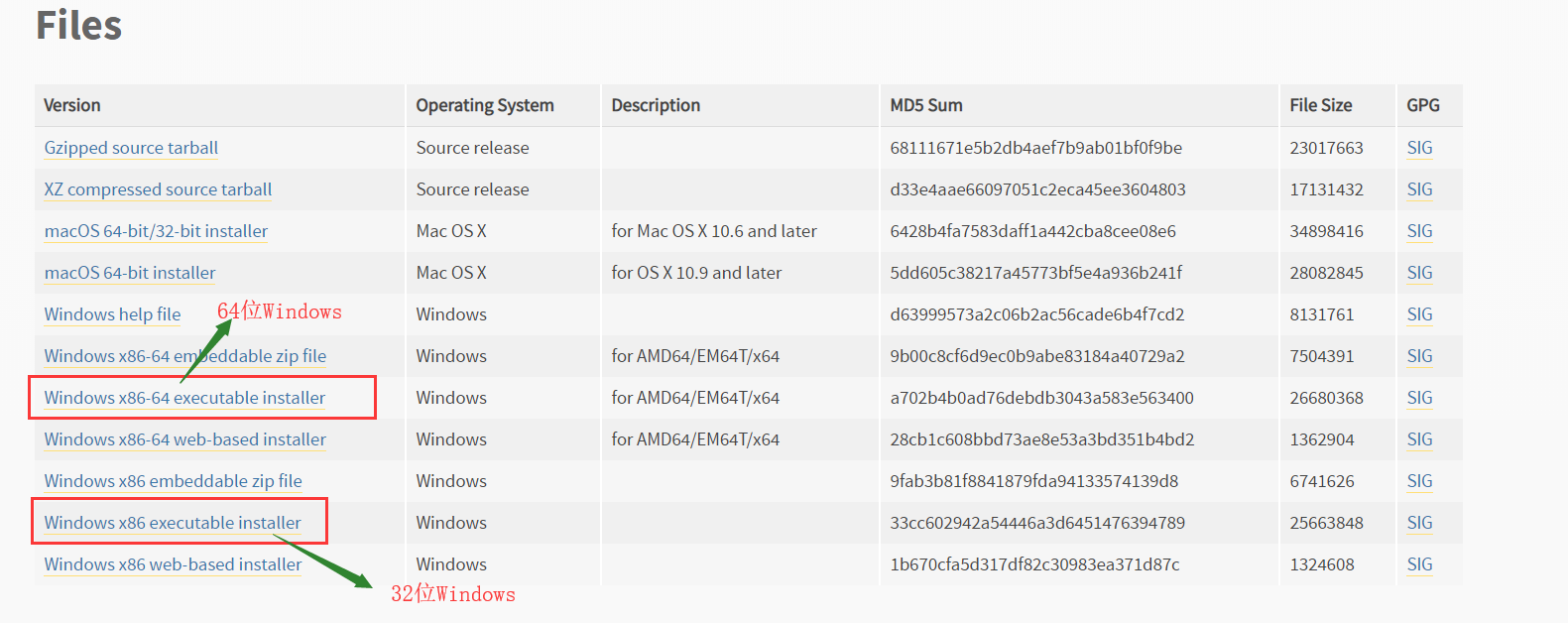下载，安装完python后：出现的四个玩意：Python 3.7 Module Docs，IDLE，Python 3.7 Manuals，Python 3.7（64-bit）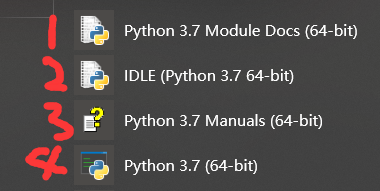Python 3.7 Module Docs（64-bit） 点击之后，会出现一个网页（将我下载的Python3.7.4文件夹中包含的模块都列了出来,页面不止这么点，还可以往下拉）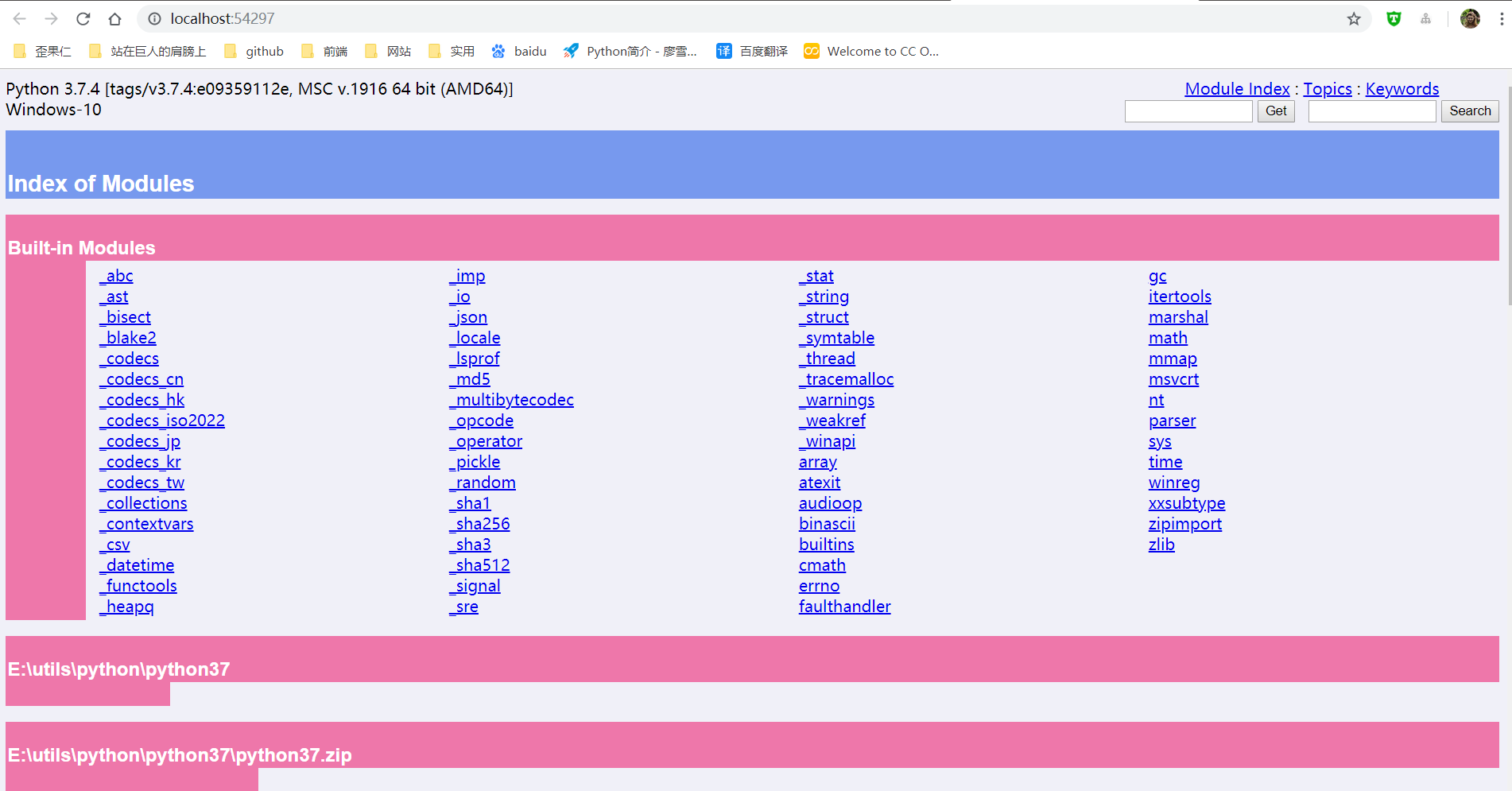IDLE（Python 3.7 64-bit） 一个Python编辑器，Python内置的集成开发工具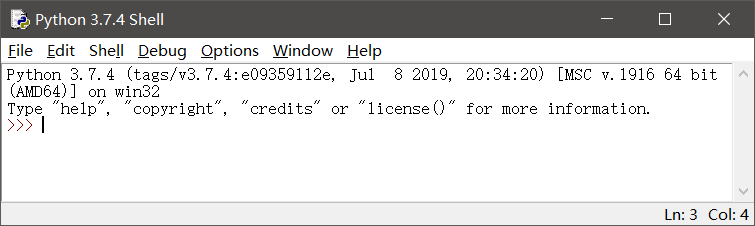Python 3.7 Manuals（64-bit） Python 3.7 开发手册  Python 3.7（64-bit） 控制台中运行Python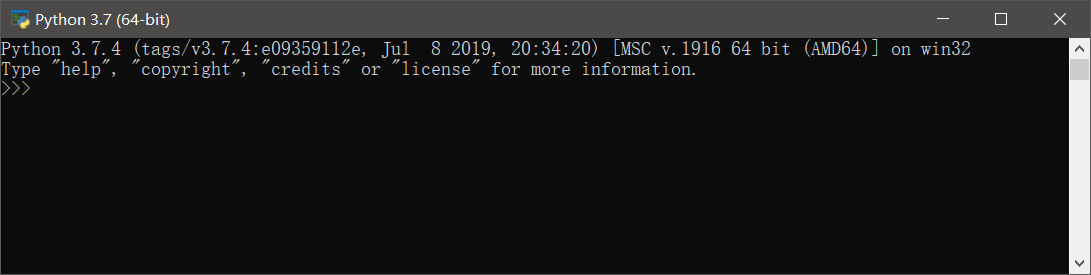二：更新pip和setuptools包，安装NumPy包，安装Matplotlib包
以下三个命令都是在控制台（windows中的cmd）中运行
更新pip和setuptools包
pip用于安装和管理Python扩展包setuptools用于发布Python包
python -m pip install -U pip setuptools

安装NumPy
Python扩展模块NumPy提供了数组和矩阵处理，以及傅立叶变换等高效的数值处理功能
 python -m pip install NumPy

安装Matplotlib包
Matplotlib是Python最著名的绘图库之一，提供了一整套和MATLAB相似的命令API，既适合交互式地进行制图，也可以作为绘图控件方便地嵌入到GUI应用程序中
python -m pip install Matplotlib

三：使用IDLE打开和执行Python源文件程序
首先： 有一个.py文件test.py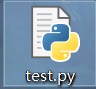使用IDLE打开.py文件的两种方式：
右键test.py---->Edit With IDLE---->Edit With IDLE 3.7（64-bit）打开IDLE，然后File---->Open（或者ctrl+O）选择.py文件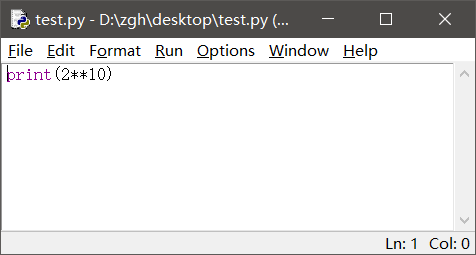运行
Run---->Run Module（或者F5） 就会出现这个界面，执行结果显示在这个界面中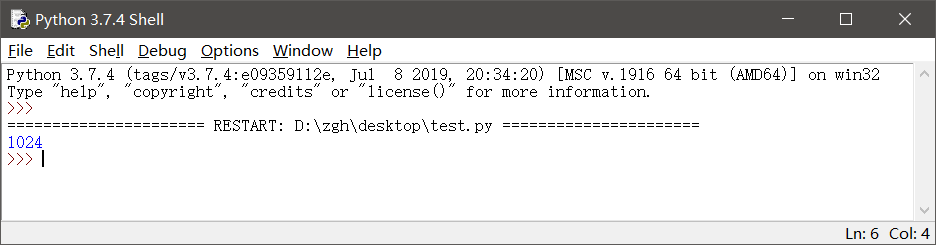补充一点： 如果在IDLE中编辑.py文件，记得修改后要保存（ctrl+s），再运行（F5）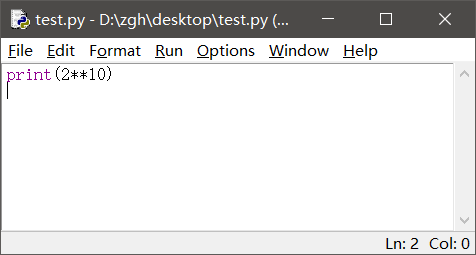四：使用资源管理器运行hello.py
hello.py文件在桌面
import random

print("hello,Python")
print("你今天的随机数字是：",random.choice(range(10)))#输出在0-9之间随机选择的整数
input()

在桌面打开PowerShell（还有两种输入方式：python hello.py或者.\hello.py）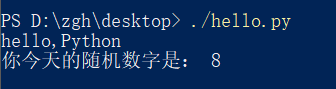或者在桌面打开cmd, 就输入hello.py或者python hello.py
补充：上述两种命令中的hello.py都是相对路径，因为文件在桌面，而且我是在桌面打开cmd，所以文件路劲可以这么简简单单的写。如果文件存储位置和cmd打开位置不一样，请使用绝对路径
五：命令行参数示例hello_argv.py
hello_argv.py文件在桌面
import sys

print("Hello,",sys.argv)
#这样写也行：
#print("Hello,"+sys.argv)

在桌面打开PowerShell（还有两种输入方式：python hello_argv.py 任意输入或者./hello_argv.py 任意输入）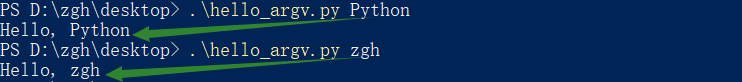或者在桌面打开cmd，就输入hello_argv.py 任意输入或者python hello_argv.py 任意输入
补充：以图中第一个命令举例，hello_argv.py即sys.argv；Python即sys.argv
第二章 Python语言基础

选择题：1、3、7、8
1. 在Python中，以下标识符合法的是
A. _B. 3CC. it’sB. str
答案：A

标识符的第一个字符必须是字母，下划线（_）；其后的字符可以是字母、下划线或数字。一些特殊的名称，作为python语言的保留关键字，不能作为标识符以双下划线开始和结束的名称通常具有特殊的含义。例如__init__为类的构造函数，一般应避免使用

B：以数字开头，错误 C：使用了'，不是字母、下划线或数字 D：str是保留关键字
3. 在下列Python语句中非法的是
A. x = y =1B. x = (y =1)C. x,y = y,xB. x=1;y=1
答案：B，C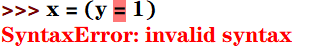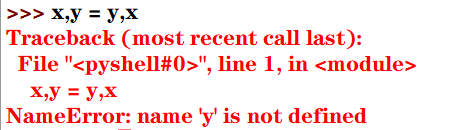7. 为了给整型变量x，y，z赋初值10，下面Python赋值语句正确的是
A. xyz=10B. x=10 y=10 z=10C. x=y=z=10B. x=10，y=10，z=10
答案：C

分号;用于在一行书写多个语句python支持链式赋值

A：赋值对象是xyz B：分号;用于在一行书写多个语句，而不是' '（即空格） D：分号;用于在一行书写多个语句，而不是,
8. 为了给整型变量x，y，z赋初值5，下面Python赋值语句正确的是
A. x=5;y=5;z=5B. xyz=5C. x,y,z=10B. x=10，y=10，z=10
答案：A

Pytho能支持序列解包赋值，但是变量的个数必须与序列的元素个数一致，否则会报错

B：赋值对象是xyz C：序列解包赋值，变量的个数必须与序列的元素个数一致，否则会报错 D：分号;用于在一行书写多个语句，而不是,
思考题：9
9.下列Python语句的输出结果是
def f():pass
print(type(f()))

结果：<class 'NoneType'>

NoneType数据类型包含唯一值None，主要用于表示空值，如没有返回值的函数的结果

上机实践：2~6
2. 编写程序，输入本金、年利率和年数，计算复利（结果保留两位小数）
money = int(input("请输入本金："))
rate = float(input("请输入年利率："))
years = int(input("请输入年数："))
amount = money*((1+rate/100)**years)
print(str.format("本金利率和为：{0:2.2f}",amount))

运行：
请输入本金：1000
请输入年利率：6.6
请输入年数：10
本金利率和为：1894.84

3. 编写程序，输入球的半径，计算球的表面积和体积（结果保留两位小数）
import math
r = float(input("请输入球的半径："))
area = 4 * math.pi * r**2
volume = 4/3*math.pi*r**3
print(str.format("球的表面积为：{0:2.2f}，体积为：{1:2.2f}",area,volume))

运行：
请输入球的半径：666
球的表面积为：5573889.08，体积为：1237403376.70

4. 编写程序，声明函数getValue(b,r,n)，根据本金b，年利率r和年数n计算最终收益v
money = int(input("请输入本金："))
rate = float(input("请输入年利率(<1)："))
years = int(input("请输入年数："))

def getValue(b,r,n):
return b*(1+r)**n

print(str.format("本金利率和为：{0:2.2f}",getValue(money,rate,years)))

运行：
请输入本金：10000
请输入年利率(<1)：0.6
请输入年数：6
本金利率和为：167772.16

5. 编写程序，求解一元二次方程x2-10x+16=0
from math import sqrt
x = (10+sqrt(10*10-4*16))/2
y = (10-sqrt(10*10-4*16))/2
print(str.format("x*x-10*x+16=0的解为：{0:2.2f}，{1:2.2f}",x,y))

运行：
x*x-10*x+16=0的解为：8.00，2.00

6. 编写程序，提示输入姓名和出生年份，输出姓名和年龄
import datetime
sName = str(input("请输入您的姓名："))
birthday = int(input("请输入您的出生年份："))
age = datetime.date.today().year - birthday
print("您好！{0}。您{1}岁。".format(sName,age))

运行：
请输入您的姓名：zgh
请输入您的出生年份：1999
您好！zgh。您20岁。

案例研究：使用Pillow库处理图像文件
https://blog.csdn.net/Zhangguohao666/article/details/102060722
通过此案例，进一步了解Python的基本概念：模块、对象、方法和函数的使用
第三章 程序流程控制

几个例题
一：编程判断某一年是否为闰年

闰年：年份能被4整除但不能被100整除，或者可以被400整除。 口诀：四年一闰，百年不闰，四百必闰

代码一：
y = int(input("请输入要判断的年份："))
if((y % 4 == 0 and y % 100 != 0) or y % 400 == 0):
print("是闰年")
else:
print("不是闰年")

代码二（使用calendar模块的isleap()函数来判断）：
from calendar import isleap

y = int(input("请输入要判断的年份："))
if(isleap(y)):print("闰年")
else:print("不是闰年")

二：利用嵌套循环打印九九乘法表
九九乘法表：
for i in range(1,10):
s = ""
for j in range(1,10):
s += str.format("%d * %d = %02d  " %(i, j, i*j))
print(s)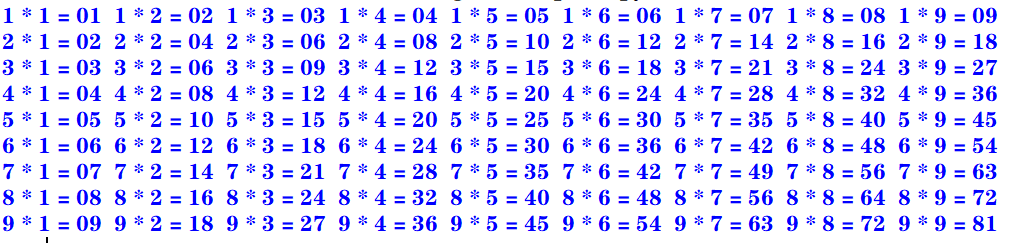下三角：
for i in range(1,10):
s = ""
for j in range(1,i+1):
s += str.format("%d * %d = %02d  " %(i, j, i*j))
print(s)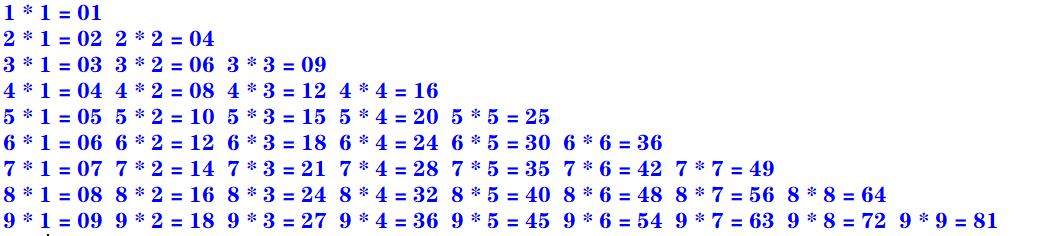上三角：
for i in range(1,10):
s = ""
for k in range(1,i):
s += "                   "
for j in range(i,10):
s += str.format("%d * %d = %02d  " %(i, j, i*j))
print(s)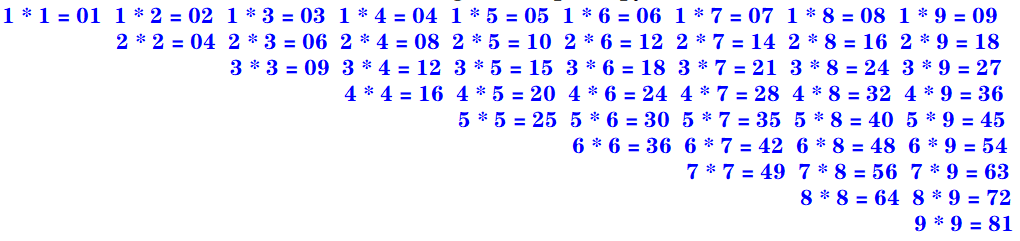三：enumerate()函数和下标元素循环示例

Python语言中的for循环直接迭代对象集合中的元素，如果需要在循环中使用索引下标访问集合元素，则可以使用内置的enumerate()函数

enumerate()函数用于将一个可遍历的数据对象（例如列表、元组或字符串）组合为一个索引序列，并返回一个可迭代对象，故在for循环当中可直接迭代下标和元素
seasons = ["Spring","Summer","Autumn","Winter"]
for i,s in enumerate(seasons,start=1):    #start默认从0开始
print("第{0}个季节：{1}".format(i,s))

运行：
第1个季节：Spring
第2个季节：Summer
第3个季节：Autumn
第4个季节：Winter

四：zip()函数和并行循环示例

如果需要并行遍历多个可迭代对象，则可以使用Python的内置函数zip()

zip()函数将多个可迭代对象中对应的元素打包成一个个元组，然后返回一个可迭代对象。如果元素的个数不一致，则返回列表的长度与最短的对象相同。
利用运算符*还可以实现将元组解压为列表
evens = [0,2,4,6,8]
odds = [1,3,5,7,9]
for e,o in zip(evens,odds):
print("{0} * {1} = {2}".format(e,o,e*o))

运行：
0 * 1 = 0
2 * 3 = 6
4 * 5 = 20
6 * 7 = 42
8 * 9 = 72

五：map()函数和循环示例

如果需要遍历可迭代对象，并使用指定函数处理对应的元素，则可以使用Python的内置函数map()

map(func,seq1[,seq2,...])

func作用于seq中的每一个元素，并将所有的调用结果作为可迭代对象返回。如果func为None，该函数的作用等同于zip()函数
计算绝对值：
>>> list(map(abs, [-1, 0, 7, -8]))
[1, 0, 7, 8]

计算乘幂：
>>> list(map(pow, range(5), range(5)))
[1, 1, 4, 27, 256]

计算ASCII码：
>>> list(map(ord, 'zgh'))
[122, 103, 104]

字符串拼接（使用了匿名函数lambda）：
>>> list(map(lambda x, y: x+y, 'zgh', '666'))
['z6', 'g6', 'h6']

选择题：1、2、3
1. 下面的Python循环体的执行次数与其他不同的是
A.
i = 0
while(i <= 10):
print(i)
i = i + 1

B.
i = 10
while(i > 0):
print(i)
i = i - 1

C.
for i in range(10):
print(i)

D.
for i in range(10,0,-1):
print(i)

答案：A
A：[0,10] 执行11次 B：[10,1] 执行10次 C：[0,9) 执行10次 D：[10,0) 执行10次
2. 执行下列Python语句将产生的结果是
x = 2; y = 2.0
if(x == y): print("Equal")
else: print("Not Equal")

A. EqualB. Not EqualC. 编译错误D. 运行时错误
答案：A

Python中的自动类型转换：
自动类型转换注意针对Number数据类型来说的当2个不同类型的数据进行运算的时候,默认向更高精度转换数据类型精度从低到高:bool int float complex关于bool类型的两个值：True 转化成整型是1；False 转化成整型是0

int类型的2转化为float类型的2.0
3. 执行下列Python语句将产生的结果是
i= 1
if(i): print(True)
else: print(False)

A. 输出1B. 输出TrueC. 输出FalseD. 编译错误
答案：B
在Python中，条件表达式最后被评价为bool值True或False。
如果表达式的结果为数值类型（0），空字符串（""），空元组（()），空列表（[]），空字典（{}），其bool值为False，否则其bool值为True
填空题：6
6. 要使语句for i in range(_,-4,-2)循环执行15次，则循环变量i的初值应当为
答案：26或者25
一开始我给的答案是26，经过评论区 亻的提醒：>>> a = 0
>>> for i in range(26, -4, -2): a+=1

>>> print(a)
15

>>> a = 0
>>> for i in range(25, -4, -2): a+=1

>>> print(a)
15

这种题目有一个规律：for i in range(x,y,z): 若循环中没有break或者continue语句， 执行次数的绝对值：result = (x-y)÷z
但实际上没有这么简单：
如果步长为 -1或者1，那么答案只有一个如果步长为 -2或者2，那么答案有两个如果步长为 -3或者3，那么答案有三个…
通过公式算出 x 之后，
如果步长为2，还要计算 (x ± 1) - z × (result-1) 的值，然后再经过琐碎的判断即可如果步长为3，还要计算 (x ± 2) - z × (result-1) 的值，……
虽然看着麻烦，但实际上是很好理解的
思考题：3~6
3. 阅读下面的Python程序，请问程序的功能是什么？
from math import sqrt

n = 0
for m in range(101,201,2):
k = int(sqrt(m))
for i in range(2, k+2):
if m % i == 0:break
if i == k + 1:
if n % 10 == 0:print()
print('%d' % m,end = " ")
n += 1

输出101到200之间的素数 每行输出10个，多余换行
运行：
101 103 107 109 113 127 131 137 139 149
151 157 163 167 173 179 181 191 193 197
199


素数（质数）是指在大于1的自然数中，除了1和它本身以外不再有其他因数的自然数。

4. 阅读下面的Python程序，请问输出的结果使什么？
n = int(input("请输入图形的行数："))
for i in range(0, n):
for j in range(0, 10 - i):print(" ", end=" ")
for k in range(0, 2 * i + 1):print(" * ", end=" ")
print("\n")

输出的是一个金字塔
运行：
请输入图形的行数：4
*

*   *   *

*   *   *   *   *

*   *   *   *   *   *   *

5. 阅读下面的Python程序，请问输出的结果使什么？程序的功能是什么？
for i in range(100,1000):
n1 = i // 100
n2 = i // 10 % 10
n3 = i % 10
if(pow(n1, 3) + pow(n2, 3) + pow(n3, 3) == i):print(i, end=" ")

输出三位数中所有的水仙花数
运行：
153 370 371 407


水仙花数 是指一个 3 位数，它的每个位上的数字的 3次幂之和等于它本身

6. 阅读下面的Python程序，请问输出的结果使什么？程序的功能是什么？
for n in range(1,1001):
total = 0; factors = []
for i in range(1, n):
if(n % i == 0):
factors.append(i)
total += i
if(total == n):print("{0} : {1}".format(n, factors))

输出1到1000的所有完数，并输出每个完数的所有因子
运行：
6 : [1, 2, 3]
28 : [1, 2, 4, 7, 14]
496 : [1, 2, 4, 8, 16, 31, 62, 124, 248]


完数 所有的真因子（即除了自身以外的约数）的和（即因子函数），恰好等于它本身

上机实践：2~14
2. 编写程序，计算1=2+3+…+100之和
使用for循环（递增）：
total = 0
for i in range(101):
total += i
print(total)

使用求和公式：
>>> (1 + 100) * 100 /2
5050.0

使用累计迭代器itertools.accumulate
>>> import itertools
>>> list(itertools.accumulate(range(1, 101)))
5050

3. 编写程序，计算10+9+8+…+1之和
使用for循环（递增）：
total = 0
for i in range(11):
total += i
print(total)

使用for循环（递减）：
total = 0
for i in range(10,0,-1):
total += i
print(total)

使用求和公式：
>>> (1 + 10) * 10 / 2
55.0

使用累计迭代器itertools.accumulate
>>> import itertools
>>> list(itertools.accumulate(range(1,11)))
55

4. 编写程序，计算1+3+5+7+…+99之和
使用for循环（递增）：
total = 0
for i in range(1,100,2):
total += i
print(total)

使用求和公式：
>>> (1 + 99) * 50 /2
2500.0

使用累计迭代器itertools.accumulate
>>> import itertools
>>> list(itertools.accumulate(range(1,100,2)))
2500

5. 编写程序，计算2+4+6+8+…+100之和
使用for循环（递增）：
total = 0
for i in range(2,101,2):
total += i
print(total)

使用求和公式：
>>> (2 + 100) * 50 / 2
2550.0

使用累计迭代器itertools.accumulate
>>> import itertools
>>> x = list(itertools.accumulate(range(2,101,2)))
>>> x[len(x)-1]
2550

6. 编写程序，使用不同的实现方法输出2000~3000的所有闰年
代码一：
for y in range(2000,3001):
if((y % 4 == 0 and y % 100 != 0) or y % 400 == 0):
print(y,end = ' ')

代码二（使用calendar模块的isleap()函数来判断）：
from calendar import isleap

for y in range(2000,3001):
if(isleap(y)):print(y,end = " ")

运行：
2000 2004 2008 2012 2016 2020 2024 2028 2032 2036 2040 2044 2048 2052 2056 2060 2064 2068 2072 2076 2080 2084 2088 2092 2096 2104 2108 2112 2116 2120 2124 2128 2132 2136 2140 2144 2148 2152 2156 2160 2164 2168 2172 2176 2180 2184 2188 2192 2196 2204 2208 2212 2216 2220 2224 2228 2232 2236 2240 2244 2248 2252 2256 2260 2264 2268 2272 2276 2280 2284 2288 2292 2296 2304 2308 2312 2316 2320 2324 2328 2332 2336 2340 2344 2348 2352 2356 2360 2364 2368 2372 2376 2380 2384 2388 2392 2396 2400 2404 2408 2412 2416 2420 2424 2428 2432 2436 2440 2444 2448 2452 2456 2460 2464 2468 2472 2476 2480 2484 2488 2492 2496 2504 2508 2512 2516 2520 2524 2528 2532 2536 2540 2544 2548 2552 2556 2560 2564 2568 2572 2576 2580 2584 2588 2592 2596 2604 2608 2612 2616 2620 2624 2628 2632 2636 2640 2644 2648 2652 2656 2660 2664 2668 2672 2676 2680 2684 2688 2692 2696 2704 2708 2712 2716 2720 2724 2728 2732 2736 2740 2744 2748 2752 2756 2760 2764 2768 2772 2776 2780 2784 2788 2792 2796 2800 2804 2808 2812 2816 2820 2824 2828 2832 2836 2840 2844 2848 2852 2856 2860 2864 2868 2872 2876 2880 2884 2888 2892 2896 2904 2908 2912 2916 2920 2924 2928 2932 2936 2940 2944 2948 2952 2956 2960 2964 2968 2972 2976 2980 2984 2988 2992 2996

7. 编写程序，计算Sn=1-3+5-7+9-11…
代码一：
n = int(input("项数："))
total = 0
flag = True
for i in range(1,2*n,2):
if(flag):
total += i
flag = False
else:
total -= i
flag = True
print(total)

代码二：
n = int(input("项数："))
total = 0
x = 2
for i in range(1,2*n,2):
total += pow(-1,x)*i
x += 1
print(total)

运行：
项数：10
-10

8. 编写程序，计算Sn=1+1/2+1/3+…
n = int(input("项数："))
total = 0.0
for i in range(1,n+1):
total += 1/i
print(total)

运行：
项数：10
2.9289682539682538

9. 编写程序，打印九九乘法表。要求输入九九乘法表的各种显示效果（上三角，下三角，矩形块等方式）
矩形块：
for i in range(1,10):
s = ""
for j in range(1,10):
s += str.format("%d * %d = %02d  " %(i, j, i*j))
print(s)下三角：
for i in range(1,10):
s = ""
for j in range(1,i+1):
s += str.format("%d * %d = %02d  " %(i, j, i*j))
print(s)上三角：
for i in range(1,10):
s = ""
for k in range(1,i):
s += "                   "
for j in range(i,10):
s += str.format("%d * %d = %02d  " %(i, j, i*j))
print(s)10. 编写程序，输入三角形的三条边，先判断是否可以构成三角形，如果可以，则进一步求三角形的周长和面积，否则报错“无法构成三角形！”
from math import sqrt

a = float(input("请输入三角形的边长a："))
b = float(input("请输入三角形的边长b："))
c = float(input("请输入三角形的边长c："))

if(a < b): a,b = b,a
if(a < c): a,c = c,a
if(b < c): b,c = c,b

if(a < 0 or b < 0 or c < 0 or b+c <= a): print("无法构成三角形！")
else:
h = (a+b+c)/2
area = sqrt(h*(h-a)*(h-b)*(h-c))
print("周长：{0}，面积：{1}".format(a+b+c,area))

运行：
请输入三角形的边长a：4
请输入三角形的边长b：3
请输入三角形的边长c：5
周长：12.0，面积：6.0

11. 编写程序，输入x，根据如下公式计算分段函数y的值。请分别用单分支语句，双分支语句结构以及条件运算语句等方法实现
y = (x2-3x)/(x+1) + 2π + sinx （x≥0 ） y = ln(-5x) + 6√(|x|+e4) - (x+1)3 (x<0)
单分支语句：
import math

x = float(input("请输入x："))
if(x >= 0):
y = (x*x - 3*x)/(x+1) + 2*math.pi + math.sin(x)
if(x < 0):
y = math.log(-5*x) + 6 * math.sqrt(abs(x) + math.exp(4)) - pow(x+1,3)

print(y)


双分支语句：
import math

x = float(input("请输入x："))
if(x >= 0):
y = (x*x - 3*x)/(x+1) + 2*math.pi + math.sin(x)
else:
y = math.log(-5*x) + 6 * math.sqrt(abs(x) + math.exp(4)) - pow(x+1,3)

print(y)

条件运算语句：
import math

x = float(input("请输入x："))
y = ((x*x - 3*x)/(x+1) + 2*math.pi + math.sin(x)) if(x >= 0) \
else (math.log(-5*x) + 6 * math.sqrt(abs(x) + math.exp(4)) - pow(x+1,3))

print(y)

运行一：
请输入x：666
668.2715406628656

运行二：
请输入x：-666
294079794.1744833

12. 编写程序，输入一元二次方程的3个系数a、b、c，求ax2+bx+c=0方程的解
import math

a = float(input("请输入系数a："))
b = float(input("请输入系数b："))
c = float(input("请输入系数c："))

delta = b*b -4*a*c

if(a == 0):
if(b == 0): print("无解")
else: print("有一个实根：",-1*c/b)
elif(delta == 0): print("有两个相等实根：x1 = x2 = ", (-1*b)/(2*a))
elif(delta > 0): print("有两个不等实根：x1 = {0}，x2 = {1}".format\
((-1*b +math.sqrt(delta))/2*a,(-1*b -math.sqrt(delta))/2*a))
elif(delta < 0): print("有两个共轭复根：x1 = {0}，x2 = {1}".format\
(complex( (-1*b)/(2*a),math.sqrt(delta*-1)/(2*a)),complex( (-1*b)/(2*a),-1*math.sqrt(delta*-1)/(2*a))))

运行一：
请输入系数a：0
请输入系数b：0
请输入系数c：10
无解

运行二：
请输入系数a：0
请输入系数b：10
请输入系数c：5
有一个实根： -0.5

运行三：
请输入系数a：1
请输入系数b：8
请输入系数c：16
有两个相等实根：x1 = x2 =  -4.0

运行四：
请输入系数a：1
请输入系数b：-5
请输入系数c：6
有两个不等实根：x1 = 3.0，x2 = 2.0

运行五：
请输入系数a：5
请输入系数b：2
请输入系数c：1
有两个共轭复根：x1 = (-0.2+0.4j)，x2 = (-0.2-0.4j)

13. 编写程序，输入整数n（n≥0）,分别利用for循环和while循环求n!
for循环
n = int(input("请输入n："))

if(n == 0): total = 1
if(n > 0):
total = 1
for i in range(n,0,-1):
total *= i

print(total)


while循环
n = int(input("请输入n："))

if(n == 0): total = 1
if(n > 0):
total = 1
while(n >= 1):
total *= n
n -= 1

print(total)

补充一个：使用累计迭代器itertools.accumulate
>>> import itertools, operator
>>> n = int(input('请输入n：'))
请输入n：7
>>> x = list(accumulate(range(1, n+1), operator.mul))
>>> x[len(x)-1]
5040

14. 编写程序，产生两个0~100（包含0和100）的随机整数a和b，求这两个整数的最大公约数和最小公倍数
现有知识点解决方法

import random

a = random.randint(0,100)
b = random.randint(0,100)
sum = a*b

print(a) #输出原来的a，b
print(b)

if(a < b): a,b = b,a

while(a%b != 0):
a,b = b,a%b

print("最大公约数：{0}，最小公倍数：{1}".format(b,sum/b))


补充：使用生成器（generate）函数：yield
>>> def func(a, b):
if(a < b): a,b = b,a
while(a%b != 0):
a,b = b,a%b
yield b

>>> import random
>>> if __name__ == '__main__':
a = random.randint(0,100)
b = random.randint(0,100)
sum = a*b
print(a,b)
t = list(iter(func(a, b)))
gcd = t[len(t)-1]
print("gcd = {0}, mcm = {1}".format(gcd, sum/gcd))

29 65
gcd = 1, mcm = 1885.0

补充：使用math模块中的gcd(x,y)函数
>>> import random
>>> import math
>>> if __name__ == '__main__':
a = random.randint(0,100)
b = random.randint(0,100)
sum = a*b
print(a,b)
gcd = math.gcd(a,b)
print("gcd = {0}, mcm = {1}".format(gcd, sum/gcd))

29 48
gcd = 1, mcm = 1392.0

案例研究：使用嵌套循环实现图像处理算法
https://blog.csdn.net/Zhangguohao666/article/details/103935185
通过图像处理算法案例，深入了解Python数据结构和基本算法流程
第四章 常用内置数据类型

几个例题
一：Python内置数据类型概述
Python中一切皆为对象，而每个对象属于某个数据类型

Python的数据类型包括：
内置的数据类型模块中定义的数据类型用户自定义的类型

四种内置的数值类型：int，float，bool，complex

int 与其他计算机语言有精度限制不同，Python中的整数位数可以为任意长度（只受限于计算机内存）。 整型对象是不可变对象。float 与其他计算机语言中的double和float对应 Python的浮点类型的精度和系统相关boolcomplex 当数值字符串中包含虚部j（或J）时即复数字面量

序列数据类型：str，tuple，bytes，list，bytearray

序列数据类型表示若干有序数据.

不可变序列数据类型：
str（字符串） 表示Unicode字符序列，例如：“zgh666” 在Python中没有独立的字符数据类型，字符即长度为1的字符串tuple（元组） 表示任意数据类型的序列，例如：(“z”,“g”,“h”,6,6,6)bytes（字节序列） 表示字节（8位）序列数据

可变序列数据类型：
list（列表） 表示可以修改的任意类型数据的序列，比如：[‘z’,‘g’,‘h’,6,6,6]bytearray（字节数组） 表示可以修改的字节（8位）数组

集合数据类型：set，frozenset

集合数据类型表示若干数据的集合，数据项目没有顺序，且不重复
set（集） 例如：{1，2，3}frozenset（不可变集）

字典数据类型：dict

字典数据类型用于表示键值对的字典 例如：{1:"zgh", 2:666}

NoneType，NotImplementedType，EllipsisType

NoneType数据类型包含唯一值None，主要用于表示空值，如没有返回值的函数的结果NotImplementedType数据类型包含唯一值NotImplemented，在进行数值运算和比较运算时，如果对象不支持，则可能返回该值EllipsisType数据类型包含唯一值Ellipsis，表示省略字符串符号...

其他数据类型

Python中一切对象都有一个数据类型，模块、类、对象、函数都属于某种数据类型 Python解释器包含内置类型， 例如： 代码对象Code objects 框架对象Frame objects 跟踪对象Traceback objects 切片对象Slice objects 静态方法对象Static method objects 类方法对象Class method objects

二：整型字面量示例

Python3.7支持使用下划线作为整数或者浮点数的千分位标记，以增强大数值的可阅读性。 二进制、八进制、十六进制则使用下划线区分4位标记

1_000_000_000  #输出1000000000

0xff_ff_ff_ff  #输出4294967295
0x_FF_FF_FF_FF  #输出4294967295

三：字符串字面量示例

两个紧邻的字符串，如果中间只有空格分隔，则自动拼接位一个字符串

'zgh' '666'  #输出'zgh666'
'zgh' + "666"   #输出'zgh666'

四：转义字符示例

转义字符后跟Unicode编码也可以表示字符
\ooo八进制Unicode码对应的字符\xhh十六进制Unicode码对应的字符

'\101'  #输出'A'
'\x41'  #输出'A'


使用r’‘或者R’'的字符串称为原始字符串，其中包含的任何字符都不进行转义

s = r'换\t行\t符\n'
s  		  #输出：'换\\t行\\t符\\n'
print(s)  #输出：换\\t行\\t符\\n

五：字符串的格式化
一：
"student number：{0}，score_average：{1}".format(2,100)
#输出：'student number：2，score_average：100'

二：
str.format("student number：{0}，score_average：{1}",2,100)
#输出：'student number：2，score_average：100'

三（兼容Python2的格式，不推荐使用）：
 "student number：%4d，score_average：%2.1f" %(2,100)
#输出：'student number：   2，score_average：100.0'

六：字符串示例，格式化输出字符串堆积的三角形

str.center()方法用于字符串两边填充str.rjust()方法用于字符串右填充

print("1".center(20))		#一行20个字符，居中对齐
print(format("121","^20"))	#一行20个字符，居中对齐
print("1".rjust(20,"*"))	#一行20个字符，右对齐，加*
print(format("121","*>20"))	#一行20个字符，右对齐，加*

运行：
         1
121
*******************1
*****************121

选择题：11
11. 关于Python字符串，下列说法错误的是
A. 字符即长度为1的字符串 B. 字符串以/0标识字符串的结束 C. 用户既可以用单引号，也可以用双引号创建字符串 D. 用三引号字符串中可以包含换行回车等特殊字符
答案：B

Python中字符串不是用\0来判断字符串结尾， 每个字符串都存有字符串的长度，通过计数来判断是否到达结尾。

虽然在c语言中\0就是来判断字符串的结尾;
填空题：4、7、8、9、10、13、21
4. Python表达式3 ** 2 ** 3的值为
答案：6561

表达式中，相同优先级的运算，从右往左

7. Python语句print(pow(-3,2),round(18.67,1),round(18.67,-1))的输出结果是
答案：9 18.7 20.0

pow()幂运算 round()四舍六入，五留双

8. Python语句print(round(123.84,0),round(123.84,-2),floor(15.5))的输出结果是
答案：124.0 100.0 15

补充：floor()是math模块中的方法，向下取整

9. Python语句print(int(‘20’,16),int(‘101’,2))的输出结果是
答案：32 5

注意：int(x,y)是指将y进制的数值x转化为10进制数

10. Python语句print(hex(16),bin(10))的输出结果是
答案：0x10 0b1010

hex(x)将十进制数x转化为十六进制，以字符串形式输出 bin(x)将十进制数x转化为二进制，以字符串形式输出

13. Python语句print(gcd(12,16),divmod(7,3))的输出结果是
答案：4 (2,1)

gcd()是math模块中的函数，求最大公约数 divmod()是内置函数，返回商和余数

21. Python语句序列 x=True;y=False;z=False;print(x or y and z) 的运行结果是
答案：True

and优先级比or高

思考题：5
5. 阅读下面的Python程序，请问输出结果是什么？
from decimal import *

ctx = getcontext()
ctx.prec = 2
print(Decimal('1.78'))#1.78
print(Decimal('1.78') + 0)#1.8
ctx.rounding = ROUND_UP
print(Decimal('1.65') + 0)#1.7
print(Decimal('1.62') + 0)#1.7
print(Decimal('-1.45') + 0)#-1.5
print(Decimal('-1.42') + 0)#-1.5
ctx.rounding = ROUND_HALF_UP
print(Decimal('1.65') + 0)#1.7
print(Decimal('1.62') + 0)#1.6
print(Decimal('-1.45') + 0)#-1.5
ctx.rounding = ROUND_HALF_DOWN
print(Decimal('1.65') + 0)#1.6
print(Decimal('-1.45') + 0)#-1.4

上机实践：2~14
2. 编写程序，格式化输出杨辉三角

杨辉三角即二项式定理的系数表，各元素满足如下条件：第一列及对角线上的元素均为1；其余每个元素等于它上一行同一列元素与前一列元素之和

我使用了一个更加精妙的规律 比如第一行为1 第二行：01 + 10 = 11 第三行：011 + 110 = 121 第四行：0121 + 1210 = 1331 。。。
def generate(numRows):
l1 = 
n = 0
while n < numRows:
print(str(l1).center(66))
l1 = [sum(t) for t in zip( + l1, l1 + )]  #利用zip函数算出每一行 如第二行 zip([0,1],[1,0])=[1,1],以此类推
n += 1
a=int(input("请输入行数"))
generate(a)

运行：
请输入行数4

[1, 1]
[1, 2, 1]
[1, 3, 3, 1]

3. 输入直角三角形的两个直角边，求三角形的周长和面积，以及两个锐角的度数。结果保留一位小数
import math

a = float(input("请输入直角三角形的直角边a："))
b = float(input("请输入直角三角形的直角边b："))
c = math.sqrt(a*a+b*b)

p = a + b + c
area = 0.5*a*b
print("三角形的周长：{0:1.1f}，面积：{1:1.1f}".format(p,area))

sina = a/c
sinb = b/c

a_degree = round(math.asin(sina) * 180 / math.pi,0)
b_degree = round(math.asin(sinb) * 180 / math.pi,0)

print("三角形直角边a的度数：{0}，b的度数：{1}".format(a_degree,b_degree))

运行：
请输入直角三角形的直角边a：3
请输入直角三角形的直角边b：4
三角形的周长：12.0，面积：6.0
三角形直角边a的度数：37.0，b的度数：53.0

4. 编程产生0~100（包含0和100）的三个随机数a、b、c，要求至少使用两种不同的方法，将三个数按从小到大的顺序排序
方法一：
import random

a = random.randint(0, 100)
b = random.randint(0, 100)
c = random.randint(0, 100)

print(str.format("原始值：{0}，{1}，{2}", a, b, c))

if(a > b): a,b = b,a
if(a > c): a,c = c,a
if(b > c): b,c = c,b

print(str.format("增序：{0}，{1}，{2}", a, b, c))

方法二（使用内置函数max、min、sum）：
import random

a = random.randint(0, 100)
b = random.randint(0, 100)
c = random.randint(0, 100)

print(str.format("原始值：{0}，{1}，{2}", a, b, c))

maxx = max(a, b, c)
minx = min(a, b, c)
median = sum([a, b, c]) - minx - maxx

print(str.format("增序：{0}，{1}，{2}", minx, median, maxx))

方法三（使用内置函数sorted）：
>>> import random
>>> a = random.randint(0,100)
>>> b = random.randint(0,100)
>>> c = random.randint(0,100)
>>> print("init value: {0} , {1} , {2}".format(a,b,c))
init value: 17 , 6 , 59
>>> sorted([a,b,c])
[6, 17, 59]

5. 编程计算有固定工资收入的党员每月所缴纳的党费。
工资基数3000元及以下者，交纳工资基数的0.5% 工资基数3000~5000元者，交纳工资基数的1% 工资基数在5000~10000元者，交纳工资基数的1.5% 工资基数超过10000元者，交纳工资基数的2%
salary = float(input("请输入有固定工资收入的党员的月工资："))
if salary <= 3000: dues = salary*0.005
elif salary <= 5000: dues = salary*0.01
elif salary <= 10000: dues = salary*0.15
else: dues = salary*0.02

print("交纳党费：",dues)

运行：
请输入有固定工资收入的党员的月工资：10001
交纳党费： 200.02

6. 编程实现袖珍计算器，要求输入两个操作数和一个操作符（+、-、*、/、%），根据操作符输出运算结果。注意/和%运算符的零异常问题
a = float(input("请输入操作数（左）："))
b = float(input("请输入操作数（右）："))
operator = input("请输入操作符（+、-、*、/、%）：")

if(b == 0 and (operator == '/' or operator == '%')):
print("分母为零，异常！")
else:
if operator == '+': result = a+b
elif operator == '-': result = a-b
elif operator == '*': result = a*b
elif operator == '/': result = a/b
elif operator == '%': result = a%b
print("{0} {1} {2}= {3}：".format(a,operator,b,result))

运行：
请输入操作数（左）：10
请输入操作数（右）：5
请输入操作符（+、-、*、/、%）：+
10.0 + 5.0= 15.0：

7. 输入三角形的3条边a、b、c，判断此3边是否可以构成三角形。若能，进一步判断三角形的性质，即为等边、等腰、直角或其他三角形
a = float(input("请输入三角形的边a："))
b = float(input("请输入三角形的边b："))
c = float(input("请输入三角形的边c："))

if(a > b): a,b = b,a
if(a > c): a,c = c,a
if(b > c): b,c = c,b

result = "三角形"
if(not(a>0 and b>0 and c>0 and a+b>c)):
result = '此三边无法构成三角形'
else:
if a == b == c: result = '等边三角形'
elif(a==b or a==c or b==c): result = '等腰三角形'
elif(a*a+b*b == c*c): result = '直角三角形'

print(result)

运行：
请输入三角形的边a：3
请输入三角形的边b：4
请输入三角形的边c：5
直角三角形

8. 编程实现鸡兔同笼问题
已知在同一个笼子里共有h只鸡和兔，鸡和兔的总脚数为f，其中h和f由用户输入，求鸡和兔各有多少只？要求使用两种方法：一是求解方程；二是利用循环进行枚举测试
h = int(input("请输入总头数："))
f = int(input("请输入总脚数："))

def fun1(h,f):
rabbits = f/2-h
chicken = h-rabbits
if(chicken < 0 or rabbits < 0): return '无解'
return chicken,rabbits

def fun2(h,f):
for i in range(0,h+1):
if(2*i + 4*(h-i) == f):return i,h-i
return '无解'

if(h>0 and f>0 and f % 2 == 0):
if fun1(h,f)=='无解':
print("无解")
else:
print("方法一：鸡：{0}，兔：{1}".format(fun1(h,f),fun1(h,f)))
print("方法二：鸡：{0}，兔：{1}".format(fun2(h,f),fun2(h,f)))
else:
print('输入的数据无意义')

运行：
请输入总头数：100
请输入总脚数：100
无解

9. 输入任意实数x，计算ex的近似值，直到最后一项的绝对值小于10-6为止
ex = 1 + x + x2/2 + x3/3! + x4/4! + … + xn/n!
x = int(input("请输入任意实数："))

e = 1
i = 1
t = 1
a = 1
while(a >= 10e-6):
t *= i
a = pow(x,i)/t
e += a
i += 1

print(e)

运行：
请输入任意实数：1
2.7182815255731922

我发现了在Python中10e-6和pow(10,-6)是有差别的，将上述代码中的10e-6改为pow(10,-6)，输出结果会有细微的差别
运行：
请输入任意实数：1
2.7182818011463845

10. 输入任意实数a（a>=0），用迭代法求x=√a，要求计算的相对偏差小于10-6
求平方根的公式：
Xn+1 = 0.5(Xn + a/Xn)
import math

a = int(input("请输入任意实数a(>=0)："))

x = a / 2
y = (x + a/x) / 2

while(abs(y-x) >= pow(10,-6)):
x = y
y = (x + a/x) / 2

print(y)

运行：
请输入任意实数a(>=0)：2
1.414213562373095

11. 即有一个数，用3除余2，用5除余3，用7除余2，请问0~1000中这样的数有哪些？
我国古代有位大将，名叫韩信。他每次集合部队，只要求部下先后按1-3，1-5，1-7报数，然后再报告一下各队每次报数的余数，他就知道到了多少人。他的这种巧妙算法被人们称作“鬼谷算”，也叫“隔墙算”，或称为“韩信点兵”，外国人还称它为“中国余数定理”。
for i in range(0,1001):
if((i % 3 == 2 )and (i % 5 == 3) and (i % 7 == 2)): print(i, end="  ")

运行：
23  128  233  338  443  548  653  758  863  968

12. 一球从100米的高度自由下落，每次落地后反弹回原高度的一半，再落下。求小球在第10次落地时共经过多少米?第10次反弹多高
规律： 第一次下落时的高度：100 第二次下落时的高度（第一次反弹的高度）：50 第三次下落时的高度（第二次反弹的高度）：25 …
n = 10

h_down = 100
h_up = 0
sum = 0
for i in range(1,n+1):
sum += h_down+h_up
h_down = h_up = h_down/2

print("小球在第十次落地时共经过：{0}米，第十次反弹高度：{1}米".format(sum,h_up))

运行：
小球在第十次落地时共经过：299.609375米，第十次反弹高度：0.09765625米

13. 猴子吃桃问题
猴子第一天摘下若干个桃子，当天吃掉一半多一个；第二天接着吃了剩下的桃子的一半多一个；以后每天都吃了前一天剩下的桃子的一半多一个。到第八天发现只剩一个桃子了。请问猴子第一天共摘了多少个桃子？
这是一个递推问题
某天所剩桃子数x 后一天所剩桃子数y = x - (x/2+1) = x/2-1
则x = 2(y+1)
result = 1
for i in range(8,0,-1):
print("第{0}天桃子数：{1}".format(i,result))
result = 2*(result+1)

运行：
第8天桃子数：1
第7天桃子数：4
第6天桃子数：10
第5天桃子数：22
第4天桃子数：46
第3天桃子数：94
第2天桃子数：190
第1天桃子数：382

14. 计算Sn = 1+11+111+…+111…111（最后一项是n个1）。n是一个随机产生的1~10（包括1和10）中的正整数
import random

n = random.randint(1,10)

x = 1
s = 0
for i in range(1,n+1):
s += x
x = 10*x+1

print("n = {0}，sn = {1}".format(n,s))

运行：
n = 6，sn = 123456

random.randint(a, b)
生成指定范围内的整数范围：[a, b]
案例研究：科学计算和数据分析
https://blog.csdn.net/Zhangguohao666/article/details/103941448
通过Python科学计算和数据分析库的安装和基本使用，了解使用Python进行科学计算的基本方法
第五章 序列数据类型

几个例题
一：Python中内置的序列数据类型

元组也称为定值表，用于存储固定不变的表列表也称为表，用于存储其值可变的表字符串是包括若干字符的序列数据，支持序列数据的基本操作字节序列数据是包括若干字节的序列。Python抓取网页时返回的页面通常为utf-8编码的字节序列。

字节序列和字符串可以直接相互转换（字节编码和解码）：
>>> s1 = b'abc'
>>> s1
b'abc'
>>> s1.decode("utf-8")
abc

>>> s2 = "中国"
>>> s2.encode("utf-8")

二：序列的切片操作示例
>>> s = 'zgh666'
>>> s
'z'
>>> s
'h'
>>> s[:3]
'zgh'
>>> s[1:3]
'gh'
>>> s[3:6]
'666'
>>> s[3:55]
'666'
>>> s[::-1]
'666hgz'
>>> s[3:2]
''
>>> s[:]
'zgh666'
>>> s[::2]
'zh6'

三：序列的连接和重复操作

通过连接操作符+可以连接两个序列，形成一个新的序列对象通过重复操作符*可以重复一个序列n次连接操作符和重复操作符也支持复合赋值运算，即：+=，*=

>>> x = 'zgh'
>>> y = '666'
>>> x + y
'zgh666'
>>> x *2
'zghzgh'
>>> x += y
>>> x
'zgh666'
>>> y *= 3
>>> y
'666666666'

四：序列的成员关系操作
innot ins.count(x) x在s中出现的次数s.index(x) x在s中第一次出现的下标
>>> s = "zgh666"
>>> 'z' in s
True
>>> 'g' not in s
False
>>> s.count('6')
3
>>> s.index('6')
3

五：序列的排序操作
sorted(iterable,key=None,reverse=False)
>>> sorted(s)
[1, 3, 5, 9]
>>> sorted(s,reverse=True)
[9, 5, 3, 1]

>>> s = 'zGhZgH'
>>> sorted(s)
['G', 'H', 'Z', 'g', 'h', 'z']
>>> sorted(s,key=str.lower)
['G', 'g', 'h', 'H', 'z', 'Z']
>>> sorted(s,key=str.lower,reverse=True)
['z', 'Z', 'h', 'H', 'G', 'g']

六：序列的拆分
变量个数与序列长度相等 若变量个数与序列的元素个数不一致，将导致ValueError
>>> data = (118,'zgh',(100,100,100))
>>> sid,name,(chinese,english,math) = data
>>> sid
118
>>> name
'zgh'
>>> chinese
100
>>> english
100
>>> math
100

变量个数与序列长度不等 如果序列长度未知，可以使用*元组变量，将多个值作为元组赋值给元组变量。在一个赋值语句中，*元组变量只允许出现一次，否则将导致SyntaxError
>>> first,second,third,*middles,last = range(10)
>>> first
0
>>> second
1
>>> third
2
>>> middles
[3, 4, 5, 6, 7, 8]
>>> last
9

>>> first,*middles,last = sorted([58,60,60,100,70,70])
>>> sum(middles)/len(middles)
65.0

使用临时变量_ 如果只需要部分数据，序列的其它位置可以使用临时变量_
>>> record = ['zgh','858990471@qq.com','17354364147','15272502101']
>>> name,_,*phone = record
>>> name
'zgh'
>>> phone
['17354364147', '15272502101']

七：使用元组字面量，tuple创建元组实例对象的实例
>>> t1 = 1,2,3
>>> t1
(1, 2, 3)

>>> t2 = (4,5,6)
>>> t2
(4, 5, 6)

>>> t3 = (9,)
>>> t3
(9,)

如果元组中只有一个项目，后面的逗号不能省略。

Python解释器把(1)解释为整数1，将(1,)解释为元组

>>> t1 = tuple()
>>> t1
()

>>> t2 = tuple("zgh666")
>>> t2
('z', 'g', 'h', '6', '6', '6')

>>> t3 = tuple(['z','g','h'])
>>> t3
('z', 'g', 'h')

八：使用列表字面量，list创建列表实例对象的实例
>>> l1 = []
>>> l1
[]

>>> l2 = ['zgh666']
>>> l2
['zgh666']

>>> l3 = [(1,2,3)]
>>> l3
[(1, 2, 3)]

>>> l1 = list()
>>> l1
[]

>>> l2 = list(b'zgh666')
>>> l2
[122, 103, 104, 54, 54, 54]

>>> l3 = list(b'aAbBcC')
>>> l3
[97, 65, 98, 66, 99, 67]

补充：列表是可变对象，故用户可以改变列表对象中元素的值，也可以通过del删除某元素
九：列表解析表达式示例
使用列表解析表达式可以简单，高效地处理一个可迭代对象，并生成结果列表
>>> [(i,i**2) for i in range(10)]
[(0, 0), (1, 1), (2, 4), (3, 9), (4, 16), (5, 25), (6, 36), (7, 49), (8, 64), (9, 81)]

>>> [i for i in range(10) if i%2==0]
[0, 2, 4, 6, 8]

>>> [(x,y,x*y) for x in range(1,4) for y in range(1,4) if x>=y]
[(1, 1, 1), (2, 1, 2), (2, 2, 4), (3, 1, 3), (3, 2, 6), (3, 3, 9)]

选择题：4、5、7、11、12
4. Python语句序列“a = (1,2,3,None,(),[]);print(len(a))”的运行结果是
>>> a = (1,2,3,None,(),[])
>>> len(a)
6

5. Python语句序列“nums = set([1,2,2,3,3,3,4]);print(len(nums))”的运行结果是
>>> nums = set([1,2,2,3,3,3,4])
>>> nums
{1, 2, 3, 4}
>>> len(nums)
4

7. Python语句序列“s1=[4,5,6];s2=s1;s1=0;print(s2)”的运行结果是
Python中变量（如s1，s2）存储在栈中，存放的是地址 [4,5,6]存储在堆中
s1 = [4,5,6]即s1存储指向堆中[4,5,6]的地址 s2 = s1地址赋值，即s2和s1都指向同一个地址 所以对列表进行修改，两者的显示都会发生变化
>>> s1 = [4,5,6]
>>> s2 = s1
>>> s1 = 0
>>> s1
[4, 0, 6]
>>> s2
[4, 0, 6]

11. Python语句序列“s={‘a’,1,‘b’,2};print(s[‘b’])”的运行结果是
A. 语法错B. ‘b’C. 1D. 2
答案：A
通过值访问集合是没有意义的，语法也不支持
>>> s ={'a',1,'b',2}
>>> print(s['b'])
Traceback (most recent call last):
File "<pyshell#29>", line 1, in <module>
print(s['b'])
TypeError: 'set' object is not subscriptable

补充：集合set是无序不重复的，是无法通过下标访问的
12. Python语句print(r"\nGood")的运行结果是
A. 新行和字符串GoodB. r"\nGood"C. \nGoodD. 字符r、新行和字符串Good
答案：C
>>> print(r"\nGood")
\nGood


r""声明原始字符串

填空题：1、5、6、12、13、14
1. Python语句序列“fruits = [‘apple’,‘banana’,‘bear’];print(fruits[-1][-1])”的运行结果是
注意：fruit[-1]是字符串’bear’ 所以：fruit[-1][-1]即'bear[-1]'
>>> fruits = ['apple','banana','pear']
>>> fruits[-1]
'pear'
>>> fruits[-1][-1]
'r'

5. Python语句 print(’%d%%%d’%(3/2,3%2)) 的运行结果是
>>> print('%d%%%d'%(3/2,3%2))
1%1

6. Python语句序列“s = [1,2,3,4];s.append([5,6]);print(len(s))”的运行结果是
答案：5
注意append()和extend()函数的区别 s.append(x)将对象x追加到s尾部 s.extend(x)将序列x追加到s尾部
append
>>> s = [1,2,3,4]
>>> s.append([5,6])
>>> s
[1, 2, 3, 4, [5, 6]]
>>> len(s)
5

extend
>>> s = [1,2,3,4]
>>> s.extend([5,6])
>>> s
[1, 2, 3, 4, 5, 6]
>>> len(s)
6

12
>>> s =('a','b','c','d','e')
>>> s
'c'
>>> s[2:3]
('c',)
>>> s[2:4]
('c', 'd')
>>> s[1::2]
('b', 'd')
>>> s[-2]
'd'
>>> s[::-1]
('e', 'd', 'c', 'b', 'a')
>>> s[-2:-1]
('d',)
>>> s[-99:-5]
()
>>> s[-99:-3]
('a', 'b')
>>> s[::]
('a', 'b', 'c', 'd', 'e')
>>> s[1:-1]
('b', 'c', 'd')

13
>>> s = [1,2,3,4,5,6]
>>> s[:1] = []
>>> s
[2, 3, 4, 5, 6]

>>> s[:2] = 'a'
>>> s
['a', 4, 5, 6]

>>> s[2:] = 'b'
>>> s
['a', 4, 'b']

>>> s[2:3] = ['x','y']
>>> s
['a', 4, 'x', 'y']

>>> del s[:1]
>>> s
[4, 'x', 'y']

14
>>> s = ['a','b']
>>> s.append([1,2])
>>> s
['a', 'b', [1, 2]]
>>> s.extend('34')
>>> s
['a', 'b', [1, 2], '3', '4']
>>> s.extend([5,6])
>>> s
['a', 'b', [1, 2], '3', '4', 5, 6]
>>> s.insert(1,7)
>>> s
['a', 7, 'b', [1, 2], '3', '4', 5, 6]
>>> s.insert(10,8)
>>> s
['a', 7, 'b', [1, 2], '3', '4', 5, 6, 8]
>>> s
['a', 7, 'b', [1, 2], '3', '4', 5, 6]
>>> s.remove('b')
>>> s
['a', 7, [1, 2], '3', '4', 5, 6]
>>> s[3:] =[]
>>> s
['a', 7, [1, 2]]
>>> s.reverse()
>>> s
[[1, 2], 7, 'a']
>>>

思考题：2、3、5
2. 阅读下面的Python语句，请问输出结果是什么？
n = int(input('请输入图形的行数：'))

for i in range(n,0,-1):
print(" ".rjust(20-i),end=' ')
for j in range(2*i-1):print(" * ",end=' ')
print('\n')

for i in range(1,n):
print(" ".rjust(19-i),end=' ')
for j in range(2*i+1):print(" * ",end=' ')
print('\n')

运行一：
请输入图形的行数：1
*

运行二：
请输入图形的行数：2
*   *   *

*

*   *   *

运行三：
请输入图形的行数：3
*   *   *   *   *

*   *   *

*

*   *   *

*   *   *   *   *

3. 阅读下面的Python语句，请问输出结果是什么？
n = int(input('请输入上（或下）三角行数：'))

for i in range(0,n):
print(" ".rjust(19-i),end=' ')
for j in range(2*i+1):print(" * ",end=' ')
print('\n')

for i in range(n-1,0,-1):
print(" ".rjust(20-i),end=' ')
for j in range(2*i-1):print(" * ",end=' ')
print('\n')

运行：
请输入上（或下）三角行数：4
*

*   *   *

*   *   *   *   *

*   *   *   *   *   *   *

*   *   *   *   *

*   *   *

*

5. 阅读下面的Python语句，请问输出结果是什么？
先看这三句：
>>> names1 = ['Amy','Bob','Charlie','Daling']
>>> names2 = names1
>>> names3 = names1[:]

毫无疑问，此时names1,names2,names3的值都是[‘Amy’,‘Bob’,‘Charlie’,‘Daling’] 但是
>>> id(names1)
2338529391368
>>> id(names2)
2338529391368
>>> id(names3)
2338529391560

names1和names2指向同一个地址 而names3指向另一个地址
然后：
>>> names2 = 'Alice'
>>> names3 = 'Ben'
>>> names1
['Alice', 'Bob', 'Charlie', 'Daling']
>>> names2
['Alice', 'Bob', 'Charlie', 'Daling']
>>> names3
['Amy', 'Ben', 'Charlie', 'Daling']

最后：
>>> sum = 0
>>> for ls in(names1,names2,names3):
if ls == 'Alice': sum+=1
if ls == 'Ben':sum+=2

>>> print(sum)
4

上机实践：2~6
2. 统计所输入字符串中单词的个数，单词之间用空格分隔
s = input("请输入字符串：")

num = 0
for i in s:
if((i >= 'a' and i <= 'z') or (i >= 'A' and i <= 'Z')):
num += 1

print("其中的单词总数：",num)

运行：
请输入字符串：zgh666 ZGH6
其中的单词总数： 6

3. 编写程序，删除一个list里面重复元素
方法一：利用set集合不重复的性质（但结果不能保证原来的顺序）
l = [1,2,2,3,3,3,4,5,6,6,6]
s = set(l)
l = list(s)
print(l)

运行：
[1, 2, 3, 4, 5, 6]

方法二：既可以去除重复项，又可以保证原来的顺序
def unique(items):
items_existed = set()
for item in items:
if item not in items_existed:
yield item

if __name__ == '__main__':
a = [1, 8, 5, 1, 9, 2, 1, 10]
a1 = unique(a)
print(list(a1))


运行结果：
[1, 8, 5, 9, 2, 10]

对代码的分析：
可以看出，unique()函数返回的并不是items_existed，而是利用了yield

在函数定义中，如果使用yield语句代替return返回一个值，则定义了一个生成器函数（generator） 生成器函数是一个迭代器，是可迭代对象，支持迭代

a1 = unique(a) 这个函数返回的实际上是一个可迭代对象 若print(a1)得到的会是：<generator object unique at 0x0000016E23AF4F48>所以，要得到去掉重复后的列表的样子，需要将可迭代对象a1放在list()中 运行：
4. 编写程序，求列表[9,7,8,3,2,1,55,6]中的元素个数、最大值、最小值，以及元素之和、平均值。请思考有几种实现方法？
内置函数：
s = [9,7,8,3,2,1,55,6]

print("元素个数：{0}，最大值：{1}，最小值：{2}，和：{3}，平均值：{4}".\
format(len(s),max(s),min(s),sum(s),sum(s)/len(s)))

直接访问元素列表（for i in s…）：
s = [9,7,8,3,2,1,55,6]

sum = 0
max = s
min = s
length = 0
for i in s:
sum += i
length += 1
if(i > max): max = i
if(i < min): min = i

print("元素个数：{0}，最大值：{1}，最小值：{2}，和：{3}，平均值：{4}".\
format(length,max,min,sum,sum/length))


间接访问列表元素（for i in range(0,len(s))…）：
s = [9,7,8,3,2,1,55,6]

sum = 0
max = s
min = s
length = len(s)
for i in range(0,length):
sum += s[i]
if(s[i] > max): max = s[i]
if(s[i] < min): min = s[i]

print("元素个数：{0}，最大值：{1}，最小值：{2}，和：{3}，平均值：{4}".\
format(length,max,min,sum,sum/length))


正序访问（i=0;while i<len(s)…）：
s = [9,7,8,3,2,1,55,6]

sum = 0
max = s
min = s
length = len(s)

i = 0
while(i < length):
sum += s[i]
if(s[i] > max): max = s[i]
if(s[i] < min): min = s[i]
i += 1

print("元素个数：{0}，最大值：{1}，最小值：{2}，和：{3}，平均值：{4}".\
format(length,max,min,sum,sum/length))


反序访问（i=len(s)-1;while i>=0…）：
s = [9,7,8,3,2,1,55,6]

sum = 0
max = s
min = s
length = len(s)

i = length-1
while(i >= 0):
sum += s[i]
if(s[i] > max): max = s[i]
if(s[i] < min): min = s[i]
i -= 1

print("元素个数：{0}，最大值：{1}，最小值：{2}，和：{3}，平均值：{4}".\
format(length,max,min,sum,sum/length))


while True：…break
s = [9,7,8,3,2,1,55,6]

sum = 0
max = s
min = s
length = len(s)

i = 0
while(True):
if(i > length-1): break
sum += s[i]
if(s[i] > max): max = s[i]
if(s[i] < min): min = s[i]
i += 1

print("元素个数：{0}，最大值：{1}，最小值：{2}，和：{3}，平均值：{4}".\
format(length,max,min,sum,sum/length))

运行：
元素个数：8，最大值：55，最小值：1，和：91，平均值：11.375

5. 编写程序，将列表[9,7,8,3,2,1,5,6]中的偶数变成它的平方，奇数保持不变
l = [9,7,8,3,2,1,5,6]

for i,value in enumerate(l):
if(value % 2 == 0):l[i] = value**2

print(l)

运行：
[9, 7, 64, 3, 4, 1, 5, 36]

6. 编写程序，输入字符串，将其每个字符的ASCII码形成列表并输出
s = input("请输入一个字符串：")
l = list()
for i in s:
l.append(ord(i))

print(l)

运行：
请输入一个字符串：zgh666
[122, 103, 104, 54, 54, 54]

案例研究：猜单词游戏
https://blog.csdn.net/Zhangguohao666/article/details/103948234
通过猜单词游戏的设计和实现，帮助读者了解使用Python系列数据类型和控制流程
第六章 输入和输出

几个例题
一：运行时提示输入密码

输入密码时，一般需要不明显，则可以使用模块getpass，以保证用户输入的密码在控制台中不回显

import getpass

else:
print('failed!')

input()#为了看到输出结果。因为执行完毕后，控制台会立马关闭


注意：上面这个代码，如果使用IDLE执行，会因为安全问题而执行失败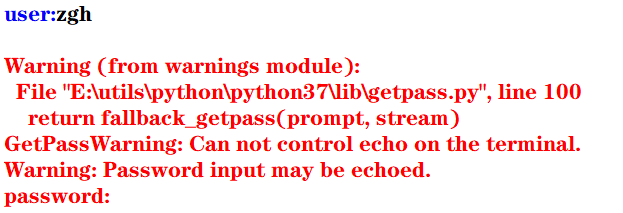但是，在控制台中执行就没问题，看输出结果（可以看到，输入的密码不会显示出来）：
user:zgh

二：重定向标准输出到一个文件的示例
这种重定向由控制台完成，而与Python本身无关。

格式： 程序 > 输出文件

其目的是将显示屏从标准输出中分离，并将输出文件与标准输出关联，即程序的执行结果将写入输出文件，而不是发送到显示屏中显示

首先准备一个test.py文件（代码如下）
import sys,random

n = int(sys.argv)
for i in range(n):
print(random.randrange(0,100))

然后在PowerShell中：python test.py 100 > scores.txt 记住，切记，一定要注意：千万能省略python，这样写./test.py 100 > scores.txt会出现问题，生成的scores文件中会没有任何内容！！！（原因未知）
然后在当前目录下，100个[0,100)范围内的的整数生成在scores.txt文件中了
三：重定向文件到标准输入

格式： 程序 < 输入文件

其目的是将控制台键盘从标准输入中分离，并将输入文件与标准输入关联，即程序从输入文件中读取输入数据，而不是从键盘中读取输入数据

准备一个average.py文件（代码如下）
import sys

total =0.0
count = 0
for line in sys.stdin:
count += 1
total += float(line)

avg = total/count
print("average:",avg)

然后问题总是不期而至， 在PowerShell中：python average.py < scores.txt，会报错，PowerShell会提示你：“<”运算符是为将来使用而保留的。 很无奈，我只能使用cmd了，然后得出结果
四：管道

格式： 程序1 | 程序2 | 程序3 | … | 程序4

其目的是将程序1的标准输出连接到程序2的标准输入， 将程序2的标准输出连接到程序3的标准输入，以此类推

例如： 打开cmd，输入python test.py 100 | average.py，其执行结果等同于上面两个例子中的命令
使用管道更加简洁，且不用创建中间文件，从而消除了输入流和输出流可以处理的数据大小的限制，执行效率更高
五：过滤器
使用操作系统实用程序more逐屏显示数据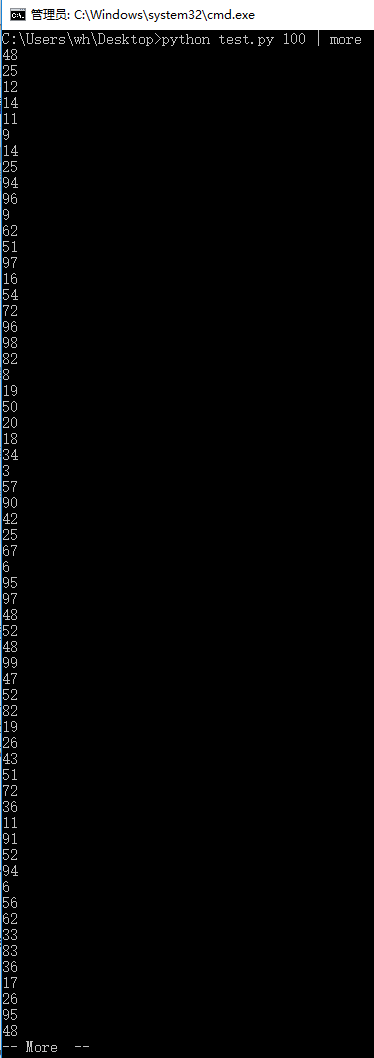使用操作系统实用程序sort排序输出数据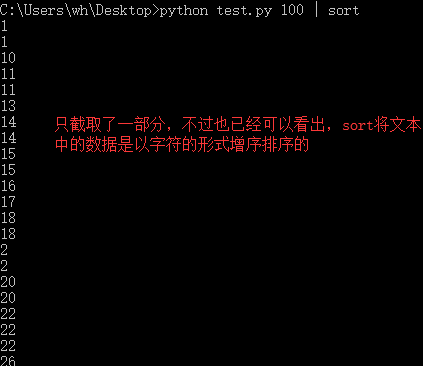more和sort都可以在一个语句中使用
填空题：1、2

print(value, ..., sep = ' ', end = '\n', file = sys.stdout, flush = False)
sep（分隔符，默认为空格）end（换行符，即输入的末尾是个啥）file（写入到指定文件流，默认为控制台sys.stdout）flush（指定是否强制写入到流）

1
>>> print(1,2,3,4,5,sep='-',end='!')
1-2-3-4-5!

2
>>> for i in range(10):
print(i,end=' ')

0 1 2 3 4 5 6 7 8 9

例题及上机实践：2~5
2. 尝试修改例6.2编写的命令行参数解析的程序，解析命令行参数所输入边长的值，计算并输出正方形的周长和面积

argparse模块用于解析命名的命令行参数，生成帮助信息的Python标准模块

例6.2：解析命令行参数所输入的长和宽的值，计算并输出长方形的面积
import argparse

parser = argparse.ArgumentParser()
parser.add_argument('--length', default = 10, type = int, help = '长度')
parser.add_argument('--width', default = 5, type = int, help = '宽度')

args = parser.parse_args()
area = args.length * args.width
print('面积 = ', area)

input()#加这一句是为了可以看到输出结果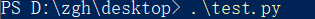输出：面积 = 50
如果在执行这个模块时，加入两个命令行参数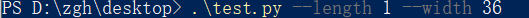输出：面积 = 36

基本上看了上面这个例子后，就可以理解argparse的用法了

本题代码：
import argparse

parser = argparse.ArgumentParser()
parser.add_argument('--length', default = 10, type = int, help = '长度')

args = parser.parse_args()
area = args.length ** 2
perimeter = 4 * args.length
print('面积 = {0}，周长 = {1}'.format(area,perimeter))

input()#加这一句是为了可以看到输出结果


在PowerShell中输入.\test.py 不给命令行参数，输出是以默认值来计算的 输出：面积 = 100，周长 = 40
给命令行参数：.\test.py --length 1 输出：面积 = 1，周长 = 4
3. 尝试修改例6.8编写读取并输出文本文件的程序，由命令行第一个参数确认所需输出的文本文件名

f = open(file, mode = 'r' , buffering = -1, encoding = None)
file是要打开或创建的文件名，如果文件不在当前路径，需指出具体路径mode是打开文件的模式，模式有： ‘r’（只读） ‘w’（写入，写入前删除就内容） ‘x’（创建新文件，如果文件存在，则导致FileExistsError） ‘a’（追加） ‘b’（二进制文件） ‘t’（文本文件，默认值） ‘+’（更新，读写）buffering表示是否使用缓存（缓存为-1，表示使用系统默认的缓冲区大小）encoding是文件的编码

例6.8：读取并输出文本文件
import sys

filename = sys.argv#就读取本文件，骚的呀皮
f = open(filename, 'r', encoding = 'utf-8')

line_no = 0
while True:
line_no += 1
if line:
print(line_no, ":", line)
else:
break
f.close()

输出（代码输出的就是本python文件）：
1 : import sys

2 :

3 : filename = sys.argv#就读取本文件，骚的呀皮

4 : f = open(filename, 'r', encoding = 'utf-8')

5 :

6 : line_no = 0

7 : while True:

8 :     line_no += 1

10 :     if line:

11 :         print(line_no, ":", line)

12 :     else:

13 :         break

14 : f.close()

15 :


本题代码：
对例题代码进行些许修改就可以了，首先将上例中的第二个语句改为：filename = sys.argv，再考虑下面怎么进行
准备一个用来测试的文件test.txt：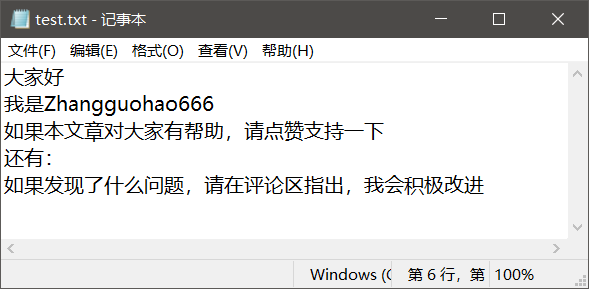对于这个文件要注意一点（你们很可能回出现这个问题！！！），win10默认创建的文本文件的字符编码是ANSI
代码怎么写，有两种：
将test.txt文本文件的编码修改为utf-8，代码如上所说 记事本方式打开test.txt文件，点击文件，点击另存为，看到下方的编码（修改为utf-8）test.txt就用默认的ANSI编码方式，再将上例代码的第三个语句修改为f = open(filename, 'r', encoding = 'ANSI')
在PowerShell中输入：./test.py test.txt 输出：
1 : ﻿大家好

2 : 我是Zhangguohao666

3 : 如果本文章对大家有帮助，请点赞支持一下

4 : 还有：

5 : 如果发现了什么问题，请在评论区指出，我会积极改进

4. 尝试修改例6.9编写利用with语句读取并输出文本文件的程序，由命令行第一个参数确认所需输出的文本文件名

为了简化操作，Python语言中与资源相关的对象可以实现上下文管理协议，可以使用with语句，确保释放资源。 with open(file,mode) as f:

例6.9：利用with语句读取并输出文本文件
import sys

filename = sys.argv

line_no = 0
with open(filename, 'r', encoding = 'utf-8') as f:
for line in f:
line_no += 1
print(line_no, ":", line)
f.close()


基本上，看这个例子，就可以上手with语句了

本题代码：
还是上一题准备的文本文件， 代码一（文本文件的编码为默认的ANSI）：
import sys

filename = sys.argv

line_no = 0
with open(filename, 'r', encoding = 'ANSI') as f:
for line in f:
line_no += 1
print(line_no, ":", line)
f.close()


代码二（将文本文件的编码修改为utf-8）：
import sys

filename = sys.argv

line_no = 0
with open(filename, 'r', encoding = 'utf-8') as f:
for line in f:
line_no += 1
print(line_no, ":", line)
f.close()


本题的输出，我再不要脸的放一次吧：
1 : ﻿大家好

2 : 我是Zhangguohao666

3 : 如果本文章对大家有帮助，请点赞支持一下

4 : 还有：

5 : 如果发现了什么问题，请在评论区指出，我会积极改进

5. 尝试修改例6.12编写标准输出流重定向的程序，从命令行第一个参数中获取n的值，然后将0-n，0-n的2倍值，2的0-n次幂的列表打印输出到out.log文件中
例6.12：从命令行第一个参数中获取n的值，然后将0-n，2的0-n次幂的列表打印输出到out.log文件中

标准输入流文件对象：sys.stdin， 默认值为sys.__stdin__标准输出流文件对象：sys.stdout， 默认值为sys.__stdout__错误输出流文件对象（标准错误流文件对象）：sys.stderr 默认值为sys.__stderr__

书中给的代码是这样的：
import sys

n = int(sys.argv)
power = 1
i = 0

f = open('out.log', 'w')
sys.stdout = f

while i <= n:
print(str(i), ' ', str(power))
power = 2*power
i += 1
sys.stdout = sys.__stdout__

如果使用的编辑器是PyCharm（现在大多数编辑器会帮你对代码进行优化和处理一些隐患），运行书中的这个代码没有问题。
但是： 若使用的编辑器是python自带的IDLE，运行这个代码有问题！
第一：out.log文件会生成，但是没有东西 （发现文件关闭不了（就是×不掉）， 确定是文件没关闭（f.close()）的原因）
第二：控制台没有输出’done’语句（估计是IDLE编辑器处理不了__stdout__这个值）
经过研究后，发现（基于IDLE编辑器）： 如果在上面的代码中加入f.close()后，该输入的东西都成功输入进out.log文件了， 但是， 还有一个问题 控制台依旧没有输出’done’语句 经过一步步的断点调试（就是手动写print） 发现sys.stdout = sys.__stdout__不会执行
然后进行改动后，就可以了，代码如下： （既然__stdout__不好使，就使用中间变量）
import sys

n = int(sys.argv)
power = 1
i = 0

output = sys.stdout
f = open('out.log', 'w')
sys.stdout = f

while i <= n:
print(str(i), ' ', str(power))
power = 2*power
i += 1

f.close()
sys.stdout = output
print('done!')#这一句是用来检测上面的代码是否成功执行



问题虽然解决，但是原因没有彻底弄清楚，求助。。。。。。

本题代码：
import sys

n = int(sys.argv)
power = 1
i = 0

output = sys.stdout
f = open('out.log', 'w')
sys.stdout = f

while i <= n:
print(str(i), ' ',  str(2*i),  ' ', str(power))
power = 2*power
i += 1

f.close()
sys.stdout = output
print('done!')#这一句是用来检测上面的代码是否成功执行


比如时输入的命令行参数是6 输出：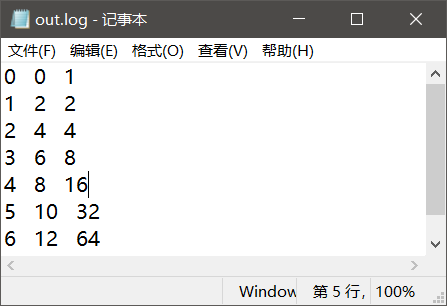案例研究：21点扑克牌游戏
https://blog.csdn.net/Zhangguohao666/article/details/103948545
通过21点扑克牌游戏的设计和实现，了解使用Python数据类型、控制流程和输入输出
第七章 错误和异常处理

Python语言采用结构化的异常处理机制捕获和处理异常

而我感觉，Python在这方面的知识点其实和Java的差不多
几个例题
一：程序的错误和异常处理
语法错误

指源代码中的拼写错误，这些错误导致Python编译器无法把Python源代码转换为字节码，故也称之为编译错误

运行时错误

在解释执行过程中产生的错误

例如：
程序中没有导入相关的模块，NameError程序中包括零除运算，ZeroDivisionError程序中试图打开不存在的文件，FileNotFoundError
逻辑错误

程序可以执行（程序运行本身不报错），但执行结果不正确。 对于逻辑错误，Python解释器无能为力，需要用户根据结果来调试判断

大部分由程序错误而产生的错误和异常一般由Python虚拟机自动抛出。另外，在程序中如果判断某种错误情况，可以创建相应的异常类的对象，并通过raise语句抛出
>>> a = -1
>>> if(a < 0): raise ValueError("数值不能为负数")

Traceback (most recent call last):
File "<pyshell#9>", line 1, in <module>
if(a < 0): raise ValueError("数值不能为负数")
ValueError: 数值不能为负数
>>>


在程序中的某个方法抛出异常后，Python虚拟机通过调用堆栈查找相应的异常捕获程序。如果找到匹配的异常捕获程序（即调用堆栈中的某函数使用try…except语句捕获处理），则执行相应的处理程序（try…except语句中匹配的except语句块）

如果堆栈中没有匹配的异常捕获程序，则Python虚拟机捕获处理异常，在控制台打印出异常的错误信息和调用堆栈，并中止程序的执行

二：try …except…else…finally
try:
可能产生异常的语句
except Exception1:
发生Exception1时执行的语句
except (Exception2,Exception3):
发生Exception2或Exception3时执行的语句
except Exception4 as e:
发生Exception4时执行的语句，Exception4的实例是e
except:
捕获其他所有异常
else:
无异常时执行的语句
finally:
不管异常发生与否都保证执行的语句

except语句可以写多个，但是要注意一点：系统是自上而下匹配发生的异常，所以用户需要将带有最具体的（即派生类程度最高的）异常类的except写在前面
三：创建自定义异常，处理应用程序中出现的负数参数的异常

自定义异常类一般继承于Exception或其子类。自定义异常类的名称一般以Error或Exception为后缀

>>> class NumberError(Exception):
def __init__(self,data):
Exception.__init__
(self,data)
self.data = data
def __str__(self):
return self.data + '：非法数值（<0）'

>>>
>>> def total(data):
total = 0
for i in data:
if i < 0: raise NumberError(str(i))
total += 1

>>>
>>> data1 = (44, 78, 90, 80, 55)
>>> print("sum: ",total(data1))
sum:  5
>>>
>>> data2 = (44, 78, 90, 80, -1)
>>> print("sum: ",total(data2))
Traceback (most recent call last):
File "<pyshell#24>", line 1, in <module>
print("sum: ",total(data2))
File "<pyshell#18>", line 4, in total
if i < 0: raise NumberError(str(i))
NumberError: -1：非法数值（<0）
>>>

四：断言处理
用户在编写程序时，在调试阶段往往需要判断代码执行过程中变量的值等信息：
用户可以使用print()函数打印输出结果也可以通过断点跟踪调试查看变量但使用断言更加灵活
assert语句和AssertionError
断言的声明：
assert <布尔表达式> 即：if __debug__: if not testexpression: raise AssertionErrorassert <布尔表达式>,<字符串表达式> 即：if __debug__: if not testexpression: raise AssertionError(data) 字符串表达式（即data）是断言失败时输出的失败消息
__debug__也是布尔值，Python解释器有两种：调试模式和优化模式
调试模式：__debug__ == True优化模式：__debug__ == False
在学习中，对于执行一个py模块（比如test.py）我们通常在cmd中这么输入python test.py，而这默认是调试模式。 如果我们要使用优化模式来禁用断言来提高程序效率，我们可以加一个运行选项-O，在控制台中这么输入python -O test.py
看一下断言的示例吧，理解一下用法：
a =int(input("a: "))
b =int(input("b: "))
assert b != 0, '除数不能为零'
c = a/b
print("a/b = ", c)

cmd出场： 输入正确数值时：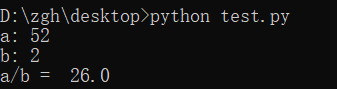输入错误数值时：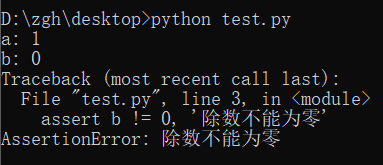禁用断言，并且输入错误数值时：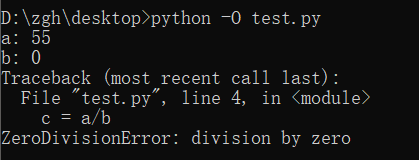案例研究：使用调试器调试Python程序
https://blog.csdn.net/Zhangguohao666/article/details/103948568
了解使用Python调试器调试程序的方法
第八章 函数和函数式编程

一些知识点总结和几个例题
Python中函数的分类：
内置函数 在程序中可以直接使用标准库函数 Python语言安装程序同时会安装若干标准库，例如math、random等第三方库函数 Python社区提供了许多其它高质量的库，在下载、安装这些库后，通过import语句可以导入库用户自定义函数
函数名为有效的标识符（命名规则为全小写字母，可以使用下划线增加可阅读性，例如my_func()）函数可以使用return返回值 如果函数体中包含return语句，则返回值， 否则不返回，即返回值为空（None），无返回值的函数相当于其它编程语言中的过程
调用函数之前程序必须先执行def语句，创建函数对象
内置函数对象会自动创建import导入模块时会执行模块中的def语句，创建模块中定义的函数Python程序结构顺序通常为import语句>函数定义>全局代码
一：产生副作用的函数，纯函数
打印等腰三角形
n = int(input("行数："))

def print_star(n):
print((" * " * n).center(50))

for i in range(1, 2*n, 2):
print_star(i)

输出：
行数：5
*
*  *  *
*  *  *  *  *
*  *  *  *  *  *  *
*  *  *  *  *  *  *  *  *

上面代码中的print_star()是一个产生副作用的函数，其副作用是向标准输出写入若干星号

副作用：例如读取键盘输入，产生输出，改变系统的状态等在一般情况下，产生副作用的函数相当于其它程序设计语言中的过程，可以省略return语句

定义计算并返回第n阶调和数（1+1/2+1/3+…+1/n）的函数，输出前n个调和数
def harmonic(n):
total = 0.0
for i in range(1, n+1):
total += 1.0/i

n = int(input("n："))

print("输出前n个调和数的值：")
for i in range(1, n+1):
print(harmonic(i))

输出：
 n：8
输出前n个调和数的值：
1.0
1.5
1.8333333333333333
2.083333333333333
2.283333333333333
2.4499999999999997
2.5928571428571425
2.7178571428571425

上面代码中的harmonic()是纯函数

纯函数：给定同样的实际参数，其返回值唯一，且不会产生其它的可观察到的副作用

注意：编写同时产生副作用和返回值的函数通常被认为是不良编程风格，但有一个例外，即读取函数。例如，input()函数既可以返回一个值，又可以产生副作用（从标准输入中读取并消耗一个字符串）
二：传递不可变对象、可变对象的引用
实际参数值默认按位置顺序依次传递给形式参数。如果参数个数不对，将会产生错误
在调用函数时：
若传递的是不可变对象（例如：int、float、bool、str对象）的引用，则如果函数体中修改对象的值，其结果实际上是创建了一个新的对象
i = 1

def func(i,n):
i += n
return i

print(i)#1
func(i,10)
print(i)#1

执行函数func()后，i依旧为1，而不是11
若传递的是可变对象（例如：list对象）的引用，则在函数体中可以直接修改对象的值
import random

def shuffle(a):
n = len(a)
for i in range(n):
r = random.randrange(i,n)
a[i],a[r] = a[r],a[i]

a = [1,2,3,4,5]
print("初始：",a)
shuffle(a)
print("调用函数后：",a)

输出：
初始： [1, 2, 3, 4, 5]
调用函数后： [1, 5, 4, 3, 2]

三：可选参数，命名参数，可变参数，强制命名参数
可选参数
在声明函数时，如果希望函数的一些参数是可选的，可以在声明函数时为这些参数指定默认值
>>> def babbles(words, times=1):
print(words * times)

>>> babbles('Hello')
Hello
>>>
>>> babbles("Hello", 2)
HelloHello
>>>

注意到一点：必须先声明没有默认值的形参，然后再声明有默认值的形参，否则报错。 这是因为在函数调用时默认是按位置传递实际参数的。
怎么理解上面那句话呢？
默认是按位置传递实际参数（如果有默认值的形参在左边，无默认值的形参在右，那么在调用函数时，你的实参该怎么传递呢？）
命名参数
位置参数：当函数调用时，实参默认按位置顺序传递形参命名参数（关键字参数）：按名称指定传入的参数 参数按名称意义明确 传递的参数与顺序无关 如果有多个可选参数，则可以选择指定某个参数值
基于期中成绩和期末成绩，按照指定的权重计算总评成绩
>>> def my_sum(mid_score, end_score, mid_rate = 0.4):
score = mid_score*mid_rate + end_score*(1-mid_rate)
print(format(score,'.2f'))

>>> my_sum(80,90)
86.00
>>> my_sum(mid_score = 80,end_score = 90)
86.00
>>> my_sum(end_score = 90,mid_score = 80)
86.00
>>>

可变参数
在声明函数时，可以通过带星号的参数（例如：def func(* param)）向函数传递可变数量的实参，调用函数时，从那一点后所有的参数被收集为一个元组在声明函数时，可以通过带双星号的参数（例如：def func(** param)）向函数传递可变数量的实参，调用函数时，从那一点后所有的参数被收集为一个字典
利用带星的参数计算各数字的累加和
>>> def my_sum(a,b,*c):
total = a+b
for i in c:
total += i

>>> print(my_sum(1,2))
3
>>> print(my_sum(1,2,3,4,5,6))
21

利用带星和带双星的参数计算各数字的累加和
>>> def my_sum(a,b,*c,**d):
total = a+b
for i in c:
total += i
for key in d:
total += d[key]

>>> print(my_sum(1,2))
3
>>> print(my_sum(1,2,3,4))
10
>>> print(my_sum(1,2,3,4,male=1,female=2))
13

强制命名参数
在带星号的参数后面声明参数会导致强制命名参数（Keyword-only），然后在调用时必须显式使用命名参数传递值因为按位置传递的参数默认收集为一个元组，传递给前面带星号的可变参数
>>> def my_sum(*, mid_score, end_score, mid_rate = 0.4):
score = mid_score*mid_rate + end_score*(1-mid_rate)
print(format(score,'.2f'))

>>> my_sum(mid_score=80,end_score=90)
86.00
>>> my_sum(end_score=90,mid_score=80)
86.00
>>> my_sum(80,90)
Traceback (most recent call last):
File "<pyshell#47>", line 1, in <module>
my_sum(80,90)
TypeError: my_sum() takes 0 positional arguments but 2 were given
>>>

四：全局语句global示例，非局部语句nonlocal示例，输出局部变量和全局变量
在函数体中可以引用全局变量，但是要为定义在函数外的全局变量赋值，需要使用global语句
pi = 2.1415926
e = 2.7182818

def my_func():
global pi
pi = 3.14
print("global pi = ", pi)
e = 2.718
print("local e = ", e)

print('module pi = ', pi)
print('module e = ', e)
my_func()
print('module pi = ', pi)
print('module e = ', e)

输出：
module pi =  2.1415926
module e =  2.7182818
global pi =  3.14
local e =  2.718
module pi =  3.14
module e =  2.7182818

在函数体中可以定义嵌套函数，在嵌套函数中如果要为定义在上级函数体的局部变量赋值，可以使用nonlocal
def outer_func():
tax_rate = 0.17
print('outer function tax rate is ',tax_rate)
def inner_func():
nonlocal tax_rate
tax_rate = 0.01
print('inner function tax rate is ',tax_rate)
inner_func()
print('outer function tax rate is ',tax_rate)

outer_func()

输出：
outer function tax rate is  0.17
inner function tax rate is  0.01
outer function tax rate is  0.01

输出局部变量和全局变量
内置函数locals()，局部变量列表内置函数globals()，全局变量列表
五：获取和设置最大递归数
在sys模块中，函数getrecursionlimit()和setrecursionlimit()用于获取和设置最大递归次数
>>> import sys
>>> sys.getrecursionlimit()
1000
>>> sys.setrecursionlimit(666)
>>> sys.getrecursionlimit()
666
>>>

六：三个有趣的内置函数：eval()、exec()、compile()
eval
对动态表达式进行求值，返回值eval(expression, globals=None, locals=None) expression是动态表达式的字符串 globals和locals是求值时使用的上下文环境的全局变量和局部变量，如果不指定，则使用当前运行上下文
>>> x = 2
>>> str_func = input("请输入表达式：")
请输入表达式：x**2+2*x+1
>>> eval(str_func)
9
>>>

exec
可以执行动态表达式，不返回值exec(str, globals=None, locals=None)
>>> exec("for i in range(10): print(i, end=' ')")
0 1 2 3 4 5 6 7 8 9
>>>

compile
编译代码为代码对象，可以提高效率compile(source, filename, mode) source为代码语句的字符串；如果是多行语句，则每一行的结尾必须有换行符\n filename为包含代码的文件 mode为编码方式，可以为'exec'（用于语句序列的执行），可以为'eval'（用于表达式求值），可以为'single'（用于单个交互语句）
>>> co = compile("for i in range(10): print(i, end=' ')", '', 'exec')
>>> exec(co)
0 1 2 3 4 5 6 7 8 9
>>>

七：map()，filter()
map(f, iterable,…)，将函数f应用于可迭代对象，返回结果为可迭代对象
示例1：
>>> def is_odd(x):
return x%2 == 1

>>> list(map(is_odd,range(5)))
[False, True, False, True, False]
>>>

示例2：
>>> list(map(abs,[1,-2,3,-4,5,-6]))
[1, 2, 3, 4, 5, 6]
>>>

示例3：
>>> list(map(str,[1,2,3,4,5]))
['1', '2', '3', '4', '5']
>>

示例4：
>>> def greater(x,y):
return x>y

>>> list(map(greater,[1,5,7,3,9],[2,8,4,6,0]))
[False, False, True, False, True]
>>>

filter(f, iterable)，将函数f应用于每个元素，然后根据返回值是True还是False决定保留还是丢弃该元素，返回结果为可迭代对象
示例1（返回个位数的奇数）：
>>> def is_odd(x):
return x%2 == 1

>>> list(filter(is_odd, range(10)))
[1, 3, 5, 7, 9]
>>>

示例2（返回三位数的回文）：
>>> list(filter(is_palindrome, range(100, 1000)))
[101, 111, 121, 131, 141, 151, 161, 171, 181, 191, 202, 212, 222, 232, 242, 252, 262, 272, 282, 292, 303, 313, 323, 333, 343, 353, 363, 373, 383, 393, 404, 414, 424, 434, 444, 454, 464, 474, 484, 494, 505, 515, 525, 535, 545, 555, 565, 575, 585, 595, 606, 616, 626, 636, 646, 656, 666, 676, 686, 696, 707, 717, 727, 737, 747, 757, 767, 777, 787, 797, 808, 818, 828, 838, 848, 858, 868, 878, 888, 898, 909, 919, 929, 939, 949, 959, 969, 979, 989, 999]
>>>

八：Lambda表达式和匿名函数

匿名函数广泛应用于需要函数对象作为参数、函数比较简单并且只使用一次的场合

格式：
lambda arg1,arg2... : <expression>

其中，arg1、arg2等为函数的参数，<expression>为函数的语句，其结果为函数的返回值
示例1（计算两数之和）：
>>> f = lambda x,y : x+y
>>> type(f)
<class 'function'>
>>> f(1,1)
2
>>>

示例2（返回奇数）：
>>> list(filter(lambda x:x%2==1, range(10)))
[1, 3, 5, 7, 9]
>>>

示例3（返回非空元素）：
>>> list(filter(lambda s:s and s.strip(), ['A', '', 'B', None, 'C', ' ']))
['A', 'B', 'C']
>>>

补充：
strip()用来去除头尾字符、空白符（\n,\r,\t,’’,即换行、回车、制表、空格）lstrip()用来去除开头字符、空白符rstrip()用来去除结尾字符、空白符
再补充一点：
\n到下一行的开头\r回到这一行的开头
示例4（返回大于0的元素）：
>>> list(filter(lambda x:x>0, [1,0,-2,8,5]))
[1, 8, 5]
>>>

示例5（返回元素的平方）：
>>> list(map(lambda x:x*x, range(10)))
[0, 1, 4, 9, 16, 25, 36, 49, 64, 81]
>>>

九：operator模块和操作符函数

Python内置操作符的函数接口，它定义了对应算术和比较等操作的函数，用于map()、filter()等需要传递函数对象作为参数的场合，可以直接使用而不需要使用函数定义或者Lambda表达式，使得代码更加简洁

示例1（concat(x,y)对应于x+y）：
>>> import operator
>>> a = 'hello'
>>>> operator.concat(a, ' world')
'hello world'

实例2（operator.gt对应于操作符>）：
>>> import operator
>>> list(map(operator.gt, [1,5,7,3,9],[2,8,4,6,0]))
[False, False, True, False, True]
>>>

十：functools.reduce()，偏函数functools.partial()，sorted()
functools.reduce()

functools.reduce(func, iterable[, iterable[, initializer]])
使用指定的带两个参数的函数func对一个数据集合的所有数据进行下列操作：使用第一个和第二个数据作为参数用func()函数运算，得到的结果再与第三个数据作为参数用func()函数运算，依此类推，最后得到一个结果可选的initialzer为初始值

示例：
>>> import functools,operator
15
25
5050
>>>
>>> functools.reduce(operator.mul, range(1,11))
3628800

偏函数functools.partial()

functools.partial(func, *arg, **keywords)
通过把一个函数的部分参数设置为默认值的方式返回一个新的可调用（callable）的partial对象主要用于设置预先已知的参数，从而减少调用时传递参数的个数

示例（2的n次方）：
>>> import functools,math
>>> pow2 = functools.partial(math.pow, 2)
>>> list(map(pow2, range(11)))
[1.0, 2.0, 4.0, 8.0, 16.0, 32.0, 64.0, 128.0, 256.0, 512.0, 1024.0]
>>>

十一：sorted()

sorted(iterable, *, key=None, reverse=False)
iterable是待排序的可迭代对象key是比较函数（默认为None，按自然顺序排序）reverse用于指定是否逆序排序

示例1（数值。默认自然排序）：
>>> sorted([1,6,4,-2,9])
[-2, 1, 4, 6, 9]
>>> sorted([1,6,4,-2,9], reverse=True)
[9, 6, 4, 1, -2]
>>> sorted([1,6,4,-2,9], key=abs)
[1, -2, 4, 6, 9]

示例2（字符串，默认按字符串字典序排序）：
>>> sorted(['Dod', 'cat', 'Rabbit'])
['Dod', 'Rabbit', 'cat']
>>> sorted(['Dod', 'cat', 'Rabbit'], key=str.lower)
['cat', 'Dod', 'Rabbit']
>>> sorted(['Dod', 'cat', 'Rabbit'], key=len)
['Dod', 'cat', 'Rabbit']

示例3（元组，默认按元组的第一个元素排序）：
>>> sorted([('Bob', 75), ('Adam', 92), ('Lisa', 88)])
[('Adam', 92), ('Bob', 75), ('Lisa', 88)]
>>> sorted([('Bob', 75), ('Adam', 92), ('Lisa', 88)], key=lambda t:t)
[('Bob', 75), ('Lisa', 88), ('Adam', 92)]

十二：函数装饰器
这玩意就很有意思了，很Java语言中的注解是很相像的
示例1：
import time,functools

def timeit(func):
def wrapper(*s):
start = time.perf_counter()
func(*s)
end = time.perf_counter()
print('运行时间：', end - start)
return wrapper

@timeit
def my_sum(n):
sum = 0
for i in range(n): sum += i
print(sum)

if __name__ == '__main__':
my_sum(10_0000)

结果：
4999950000
运行时间： 0.013929100000000028

怎么理解上面的代码呢？
首先，timeit()返回的是wrapper，而不是执行（没有小括号）@timeit相当于，在调用my_sum()的前一刻，会执行这么个语句：my_sum = timeit(my_sum)
示例2：
def makebold(fn):
def wrapper(*s):
return "<b>" + fn(*s) + "</b>"
return wrapper

def makeitalic(fn):
def wrapper(*s):
return "<i>" + fn(*s) + "</i>"
return wrapper

@makebold
@makeitalic
def htmltags(str1):
return str1

print(htmltags('Hello'))


输出：
<b><i>Hello</i></b>

选择题：1~5
1
>>> print(type(lambda:None))
<class 'function'>

2
>>> f = lambda x,y:x*y
>>> f(12, 34)
408

3
>>> f1 = lambda x:x*2
>>> f2 = lambda x:x**2
>>> print(f1(f2(2)))
8

4
>>> def f1(p, **p2):
print(type(p2))

>>> f1(1, a=2)
<class 'dict'>

5
>>> def f1(a,b,c):
print(a+b)

>>> nums = (1,2,3)
>>> f1(*nums)
3

思考题：4~11
4
>>> d = lambda p:p*2
>>> t = lambda p:p*3
>>> x = 2
>>> x = d(x)
>>> x = t(x)
>>> x = d(x)
>>> print(x)
24

5
>>> i = map(lambda x:x**2, (1,2,3))
>>> for t in i:
print(t, end=' ')

1 4 9

6
>>> def f1():
"simple function"
pass

>>> print(f1.__doc__)
simple function

7
>>> counter = 1
>>> num = 0
>>> def TestVariable():
global counter
for i in (1, 2, 3) : counter += 1
num = 10

>>> TestVariable()
>>> print(counter, num)
4 0

8
>>> def f(a,b):
if b==0 : print(a)
else : f(b, a%b)

>>> print(f(9,6))
3
None

求最大公约数
9
>>> def aFunction():
"The quick brown fox"
return 1

>>> print(aFunction.__doc__[4:9])
quick

10
>>> def judge(param1, *param2):
print(type(param2))
print(param2)

>>> judge(1, 2, 3, 4, 5)
<class 'tuple'>
(2, 3, 4, 5)

11
>>> def judge(param1, **param2):
print(type(param2))
print(param2)

>>> judge(1, a=2, b=3, c=4, d=5)
<class 'dict'>
{'a': 2, 'b': 3, 'c': 4, 'd': 5}

上机实践：2~5
2. 编写程序，定义一个求阶乘的函数fact(n)，并编写测试代码，要求输入整数n（n>=0）。请分别使用递归和非递归方式实现
递归方式：
def fact(n):
if n == 0 :
return 1
return n*fact(n-1)

n = int(input("请输入整数n（n>=0）："))
print(str(n)+" ! =  " + str(fact(n)))


非递归方式：
def fact(n):
t = 1
for i in range(1,n+1):
t *= i
return t

n = int(input("请输入整数n（n>=0）："))
print(str(n)+" ! =  " + str(fact(n)))


输出：
请输入整数n（n>=0）：5
5 ! =  120

3. 编写程序，定义一个求Fibonacci数列的函数fib(n)，并编写测试代码，输出前20项（每项宽度5个字符位置，右对齐），每行输出10个。请分别使用递归和非递归方式实现
递归方式：
def fib(n):
if (n == 1 or n == 2):
return 1
return fib(n-1)+fib(n-2)

for i in range(1,21):
print(str(fib(i)).rjust(5,' '),end = ' ')
if i %10 == 0:
print()

非递归方式：
def fib(n):
if (n == 1 or n == 2):
return 1
n1 = n2 = 1
for i  in range(3,n+1):
n3 = n1+n2
n1 = n2
n2 = n3
return n3

for i in range(1,21):
print(str(fib(i)).rjust(5,' '),end = ' ')
if i %10 == 0:
print()

输出：
    1     1     2     3     5     8    13    21    34    55
89   144   233   377   610   987  1597  2584  4181  6765

4. 编写程序，利用可变参数定义一个求任意个数数值的最小值的函数min_n(a,b,*c)，并编写测试代码。例如对于“print(min_n(8, 2))”以及“print(min_n(16, 1, 7, 4, 15))”的测试代码
def min_n(a,b,*c):
min_number = a if(a < b) else b
for n in c:
if n < min_number:
min_number = n
return min_number

print(min_n(8, 2))
print(min_n(16, 1, 7, 4, 15))

输出：
2
1

5. 编写程序，利用元组作为函数的返回值，求序列类型中的最大值、最小值和元素个数，并编写测试代码，假设测试代码数据分别为s1=[9, 7, 8, 3, 2, 1, 55, 6]、s2=[“apple”, “pear”, “melon”, “kiwi”]和s3="TheQuickBrownFox"
def func(n):
return (max(n),min(n),len(n))

s1=[9, 7, 8, 3, 2, 1, 55, 6]
s2=["apple", "pear", "melon", "kiwi"]
s3="TheQuickBrownFox"

for i in (s1,s2,s3):
print("list = ", i)
t = func(i)
print("最大值 = {0}，最小值 = {1}，元素个数 = {2}".format(t, t, t))

输出：
list =  [9, 7, 8, 3, 2, 1, 55, 6]
最大值 = 55，最小值 = 1，元素个数 = 8
list =  ['apple', 'pear', 'melon', 'kiwi']
最大值 = pear，最小值 = apple，元素个数 = 4
list =  TheQuickBrownFox
最大值 = x，最小值 = B，元素个数 = 16

案例研究：井字棋游戏
https://blog.csdn.net/Zhangguohao666/article/details/103280740
了解Python函数的定义和使用

由于本文的内容太多了，导致了两个很不好的结果， 一是：在网页中打开本篇博客的加载时间太长了，明显的卡顿很影响阅读体验； 二是：本人在对本篇文章进行更新或者修改内容时，卡的要死。 遂， 将本文第八章后面的很多内容拆分到新的文章中，望大家理解

第九章 面向对象的程序设计

《Python程序设计与算法基础教程（第二版）》江红 余青松，第九章课后习题答案
第十章 模块和客户端

《Python程序设计与算法基础教程（第二版）》江红 余青松，第十章课后习题答案
第十一章 算法与数据结构基础

《Python程序设计与算法基础教程（第二版）》江红 余青松，第十一章课后习题答案
第十二章 图形用户界面

我对图形用户界面基本无兴趣，无特殊情况，基本不打算碰这方面内容
案例研究：简易图形用户界面计算器
Python实现简易图形用户界面计算器
第十三章 图形绘制

与上一章相同，我对于图形绘制的兴趣也基本没有，尝试做了2-7题，就完全没兴趣做下去了
图形绘制模块：tkinter
2. 参考例13.2利用Canvas组件创建绘制矩形的程序，尝试改变矩形边框颜色以及填充颜色
from tkinter import *

root = Tk()
c = Canvas(root, bg = 'white', width = 130, height = 70)
c.pack()

c.create_rectangle(10, 10, 60, 60, fill = 'red')
c.create_rectangle(70, 10, 120, 60, fill = 'green', outline = 'blue', width = 5)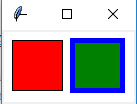创建画布对象：
root = Tk() 创建一个Tk根窗口组件rootc = Canvas(root, bg = 'white', width = 130, height = 70) 创建大小为200 * 100、背景颜色为白色的画布c.pack() 调用组件pack()方法，调整其显示位置和大小
绘制矩形：
c.create_rectangle(x0, y0, x1, y1, option, ...)

（x0，y0）是左上角的坐标（x1，y1）是右下角的坐标c.create_rectangle(70, 10, 120, 60, fill = 'green', outline = 'blue', width = 5) 用蓝色边框、绿色填充矩形，边框宽度为5
3. 参考例13.3利用Canvas组件创建绘制椭圆的程序，尝试修改椭圆边框样式、边框颜色以及填充颜色
from tkinter import *

root = Tk()
c = Canvas(root, bg = 'white', width = 280, height = 70)
c.pack()

c.create_oval(10, 10, 60, 60, fill = 'green')
c.create_oval(70, 10, 120, 60, fill = 'green', outline = 'red', width = 5)
c.create_oval(130, 25, 180, 45, dash = (10,))
c.create_oval(190, 10, 270, 50, dash = (1,), width = 2)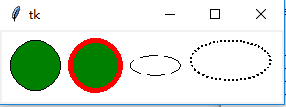绘制椭圆
c.create_oval(x0, y0, x1, y1, option, ...)

（x0，y0）是左上角的坐标（x1，y1）是右下角的坐标c.create_oval(70, 10, 120, 60, fill = 'green', outline = 'red', width = 5) 绿色填充、红色边框，宽度为5c.create_oval(130, 25, 180, 45, dash = (10,)) 虚线椭圆
4. 参考例13.4利用Canvas组件创建绘制圆弧的程序，尝试修改圆弧样式、边框颜色以及填充颜色
from tkinter import *

root = Tk()
c = Canvas(root, bg = 'white', width = 250, height = 70)
c.pack()

c.create_arc(10, 10, 60, 60, style = ARC)
c.create_arc(70, 10, 120, 60, style = CHORD)
c.create_arc(130, 10, 180, 60, style = PIESLICE)
for i in range(0, 360, 60):
c.create_arc(190, 10, 240, 60, fill = 'green', outline = 'red', start = i, extent = 30)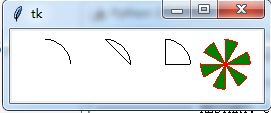绘制圆弧：
c.create_arc(x0, y0, x1, y1, option, ...)

（x0，y0）是左上角的坐标（x1，y1）是右下角的坐标选项start（开始角度，默认为0）和extend（圆弧角度，从start开始逆时针旋转，默认为90度）决定圆弧的角度范围选项start用于设置圆弧的样式
5. 参考例13.5利用Canvas组件创建绘制线条的程序，尝试修改线条样式和颜色
from tkinter import *

root = Tk()
c = Canvas(root, bg = 'white', width = 250, height = 70)
c.pack()

c.create_line(10, 10, 60, 60, arrow = BOTH, arrowshape = (3, 4, 5))
c.create_line(70, 10, 95, 10, 120, 60, fill = 'red')
c.create_line(130, 10, 180, 10, 130, 60, 180, 60, fill = 'green', width = 10, arrow = BOTH, joinstyle = MITER)
c.create_line(190, 10, 240, 10, 190, 60, 240, 60, width = 10)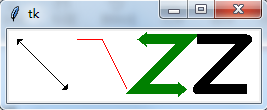绘制线条：
c.create_line(x0, y0, x1, y1, ..., xn, yn, option, ...)

（x0，y0）,（x1，y1），…，（xn，yn）是线条上各个点的坐标
6. 参考例13.6利用Canvas组件创建绘制多边形的程序，尝试修改多边形的形状、线条样式和填充颜色
from tkinter import *

root = Tk()
c = Canvas(root, bg = 'white', width = 250, height = 70)
c.pack()

c.create_polygon(35, 10, 10, 60, 60, 60, fill = 'red', outline = 'green')
c.create_polygon(70, 10, 120, 10, 120, 60, fill = 'white', outline = 'blue')
c.create_polygon(130, 10, 180, 10, 180, 60, 130, 60, outline = 'blue')
c.create_polygon(190, 10, 240, 10, 190, 60, 240, 60, fill = 'white', outline = 'black')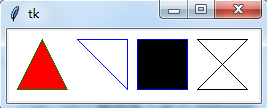绘制多边形：
c.create_polygon(x0, y0, x1, y1, ..., option, ...)

（x0，y0）,（x1，y1），…，（xn，yn）是多边形上各个顶点的坐标
7. 参考例13.7利用Canvas组件创建绘制字符串和图形的程序，绘制y = cos(x) 的图形
绘制字符串：
c.create_text(x, y, option, ...)

（x，y）是字符串放置的中心位置
y = sin(x)
from tkinter import *
import math

WIDTH, HEIGHT = 510, 210
ORIGIN_X, ORIGIN_Y = 2, HEIGHT/2 #原点

SCALE_X, SCALE_Y = 40, 100 #x轴、y轴缩放倍数
ox, oy = 0, 0
x, y = 0, 0
arc = 0 #弧度
END_ARC = 360 * 2 #函数图形画两个周期

root = Tk()
c = Canvas(root, bg = 'white', width = WIDTH, height = HEIGHT)
c.pack()

c.create_text(200, 20, text = 'y = sin(x)')
c.create_line(0, ORIGIN_Y, WIDTH, ORIGIN_Y)
c.create_line(ORIGIN_X, 0, ORIGIN_X, HEIGHT) #绘制x轴，y轴
for i in range(0, END_ARC+1, 10):
arc = math.pi * i / 180
x = ORIGIN_X + arc * SCALE_X
y = ORIGIN_Y - math.sin(arc) * SCALE_Y
c.create_line(ox, oy, x, y)
ox, oy = x, y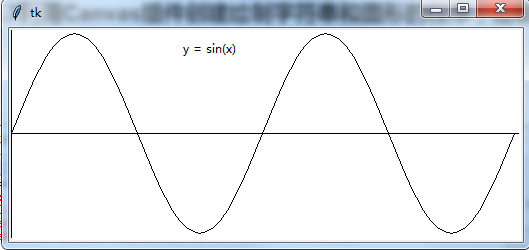y = cos(x)
from tkinter import *
import math

WIDTH, HEIGHT = 510, 210
ORIGIN_X, ORIGIN_Y = 2, HEIGHT/2 #原点

SCALE_X, SCALE_Y = 40, 100 #x轴、y轴缩放倍数
ox, oy = 0, 0
x, y = 0, 0
arc = 0 #弧度
END_ARC = 360 * 2 #函数图形画两个周期

root = Tk()
c = Canvas(root, bg = 'white', width = WIDTH, height = HEIGHT)
c.pack()

c.create_text(200, 20, text = 'y = cos(x)')
c.create_line(0, ORIGIN_Y, WIDTH, ORIGIN_Y)
c.create_line(ORIGIN_X, 0, ORIGIN_X, HEIGHT)
for i in range(0, END_ARC+1, 10):
arc = math.pi * i / 180
x = ORIGIN_X + arc * SCALE_X
y = ORIGIN_Y - math.cos(arc) * SCALE_Y
c.create_line(ox, oy, x, y)
ox, oy = x, y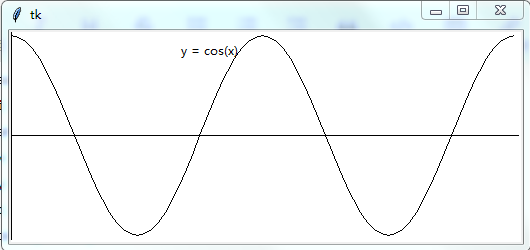图形绘制模块：turtle
…

后面章节内容：未完待续…
第十四章 数值日期和时间处理

第十五章 字符串和文本处理

第十六章 文件和数据交换

第十七章 数据访问

第十八章 网络编程和通信

第十九章 并行计算：进程、线程和协程

第二十章 系统管理
展开全文• 文章发布于公号【数智物语】 （ID：decision_engine），关注号不错过每篇干货。       转自 | 有三AI（公众号ID：yanyousan_ai） 作者 | 言有三   图像分类是计算机视觉中最基础的任务，基本上...文章发布于公号【数智物语】 （ID：decision_engine），关注公号不错过每一篇干货。

转自 | 有三AI（公众号ID：yanyousan_ai）
作者 | 言有三

图像分类是计算机视觉中最基础的任务，基本上深度学习模型的发展史就是图像分类任务提升的发展历史，不过图像分类并不是那么简单，也没有被完全解决。

01
什么是图像分类

图像分类是计算机视觉中最基础的一个任务，也是几乎所有的基准模型进行比较的任务。从最开始比较简单的10分类的灰度图像手写数字识别任务mnist，到后来更大一点的10分类的 cifar10和100分类的cifar100 任务，到后来的imagenet 任务，图像分类模型伴随着数据集的增长，一步一步提升到了今天的水平。现在，在imagenet 这样的超过1000万图像，超过2万类的数据集中，计算机的图像分类水准已经超过了人类。不过，不要把图像分类任务想的过于简单。

图像分类顾名思义就是一个模式分类问题，它的目标是将不同的图像，划分到不同的类别，实现最小的分类误差。总体来说，对于单标签的图像分类问题，它可以分为跨物种语义级别的图像分类，子类细粒度图像分类，以及实例级图像分类三大类别。

1.1
跨物种语义级别的图像分类

所谓跨物种语义级别的图像分类，它是在不同物种的层次上识别不同类别的对象，比较常见的包括如猫狗分类等。这样的图像分类，各个类别之间因为属于不同的物种或大类，往往具有较大的类间方差，而类内则具有较小的类内误差。

下面是cifar10 中的10个类别的示意图，这就是一个典型的例子。cifar包含10个类别，分别是airplane，automobile，bird，cat，deer，dog，frog，horse，ship，truck，其中airplane，automobile，ship，truck都是交通工具，bird，cat，deer，dog，frog，horse都是动物，可以认为是两个大的品类。而交通工具内部，动物内部，都是完全不同的物种，这些都是语义上完全可以区分的对象，所以cifar10的分类任务，可以看作是一个跨物种语义级别的图像分类问题。类间方差大，类内方差小。

1.2
子类细粒度图像分类

细粒度图像分类，相对于跨物种的图像分类，级别更低一些。它往往是同一个大类中的子类的分类，如不同鸟类的分类，不同狗类的分类，不同车型的分类等。

下面以不同鸟类的细粒度分类任务，加利福尼亚理工学院鸟类数据库-2011，即Caltech-UCSD Birds-200-2011为例。这是一个包含200类，11788张图像的鸟类数据集，同时每一张图提供了15个局部区域位置，1个标注框，还有语义级别的分割图。在该数据集中，以woodpecker为例，总共包含6类，即American Three toed Woodpecker，Pileated Woodpecker，Red bellied Woodpecker，Red cockaded Woodpecker，Red headed Woodpecker，Downy Woodpecker，我们取其中两类各一张示意图查看如图。从上图可以看出，两只鸟的纹理形状都很像，要想区分只能靠头部的颜色和纹理，所以要想训练出这样的分类器，就必须能够让分类器识别到这些区域，这是比跨物种语义级别的图像分类更难的问题。

1.3
实例级图像分类

如果我们要区分不同的个体，而不仅仅是物种类或者子类，那就是一个识别问题，或者说是实例级别的图像分类，最典型的任务就是人脸识别。在人脸识别任务中，需要鉴别一个人的身份，从而完成考勤等任务。人脸识别一直是计算机视觉里面的重大课题，虽然经历了几十年的发展，但仍然没有被完全解决，它的难点在于遮挡，光照，大姿态等经典难题，读者可以参考更多资料去学习。

02
图像分类模型

图像分类任务从传统的方法到基于深度学习的方法，经历了几十年的发展。这里只关注于深度学习的进展，下面重点讲述几个重要的节点。

2.1
MNIST与LeNet5

在计算机视觉分类算法的发展中，MNIST 是首个具有通用学术意义的基准。这是一个手写数字的分类标准，包含 60000 个训练数据，10000 个测试数据，图像均为灰度图，通用的版本大小为 28×28。

在上个世纪90年代末本世纪初，SVM and K-nearest neighbors方法被使用的比较多，以SVM为代表的方法，可以将MNIST分类错误率降低到了0.56%，彼时仍然超过以神经网络为代表的方法，即LeNet系列网络。LeNet网络诞生于1994年，后经过多次的迭代才有了1998年的LeNet5，是为我们所广泛知晓的版本。这是一个经典的卷积神经网络，它包含着一些重要的特性，这些特性仍然是现在CNN网络的核心。

卷积层由卷积，池化，非线性激活函数构成。从1998年至今，经过20年的发展后，卷积神经网络依然遵循着这样的设计思想。其中，卷积发展出了很多的变种，池化则逐渐被带步长的卷积完全替代，非线性激活函数更是演变出了很多的变种。
稀疏连接，也就是局部连接，这是以卷积神经网络为代表的技术能够发展至今的最大前提。利用图像的局部相似性，这一区别于传统全连接的方式，推动了整个神经网络技术的发展。

虽然LeNet5当时的错误率仍然停留在0.7%的水平，不如同时期最好的SVM方法，但随着网络结构的发展，神经网络方法很快就超过了其他所有方法，错误率也降低到了0.23%，甚至有的方法已经达到了错误率接近0的水平。

2.2
ImageNet与AlexNet

在本世纪的早期，虽然神经网络开始有复苏的迹象，但是受限于数据集的规模和硬件的发展，神经网络的训练和优化仍然是非常困难的。MNIST和CIFAR数据集都只有60000张图，这对于10分类这样的简单的任务来说，或许足够，但是如果想在工业界落地更加复杂的图像分类任务，仍然是远远不够的。

后来在李飞飞等人数年时间的整理下，2009年，ImageNet数据集发布了，并且从2010年开始每年举办一次ImageNet大规模视觉识别挑战赛，即ILSVRC。ImageNet数据集总共有1400多万幅图片，涵盖2万多个类别，在论文方法的比较中常用的是1000类的基准。

在ImageNet发布的早年里，仍然是以SVM和Boost为代表的分类方法占据优势，直到2012年AlexNet的出现。

AlexNet是第一个真正意义上的深度网络，与LeNet5的5层相比，它的层数增加了3层，网络的参数量也大大增加，输入也从28变成了224，同时GPU的面世，也使得深度学习从此进行GPU为王的训练时代。

AlexNet有以下的特点：
网络比LeNet5更深，包括5个卷积层和3个全连接层。
使用Relu激活函数，收敛很快，解决了Sigmoid在网络较深时出现的梯度弥散问题。
加入了Dropout层，防止过拟合。
使用了LRN归一化层，对局部神经元的活动创建竞争机制，抑制反馈较小的神经元放大反应大的神经元，增强了模型的泛化能力。
使用裁剪翻转等操作做数据增强，增强了模型的泛化能力。预测时使用提取图片四个角加中间五个位置并进行左右翻转一共十幅图片的方法求取平均值，这也是后面刷比赛的基本使用技巧。
分块训练，当年的GPU计算能力没有现在强大，AlexNet创新地将图像分为上下两块分别训练，然后在全连接层合并在一起。
总体的数据参数大概为240M，远大于LeNet5。

2.3
分类模型的逐年进步

2013年ILSVRC分类任务冠军网络是Clarifai，不过更为我们熟知的是zfnet。hinton的学生Zeiler和Fergus在研究中利用反卷积技术引入了神经网络的可视化，对网络的中间特征层进行了可视化，为研究人员检验不同特征激活及其与输入空间的关系成为了可能。在这个指导下对AlexNet网络进行了简单改进，包括使用了更小的卷积核和步长，将11x11的卷积核变成7x7的卷积核，将stride从4变成了2，性能超过了原始的AlexNet网络。

其中VGGNet包括16层和19层两个版本，共包含参数约为550M。全部使用3×3的卷积核和2×2的最大池化核，简化了卷积神经网络的结构。VGGNet很好的展示了如何在先前网络架构的基础上通过简单地增加网络层数和深度就可以提高网络的性能。虽然简单，但是却异常的有效，在今天，VGGNet仍然被很多的任务选为基准模型。

2015年，ResNet获得了分类任务冠军。它以3.57%的错误率表现超过了人类的识别水平，并以152层的网络架构创造了新的模型记录。由于ResNet采用了跨层连接的方式，它成功的缓解了深层神经网络中的梯度消散问题，为上千层的网络训练提供了可能。

2016年依旧诞生了许多经典的模型，包括赢得分类比赛第二名的ResNeXt，101层的ResNeXt可以达到ResNet152的精确度，却在复杂度上只有后者的一半，核心思想为分组卷积。即首先将输入通道进行分组，经过若干并行分支的非线性变换，最后合并。

在ResNet基础上，密集连接的DenseNet在前馈过程中将每一层与其他的层都连接起来。

对于每一层网络来说，前面所有网络的特征图都被作为输入，同时其特征图也都被后面的网络层作为输入所利用。

DenseNet中的密集连接还可以缓解梯度消失的问题，同时相比ResNet，可以更强化特征传播和特征的复用，并减少了参数的数目。DenseNet相较于ResNet所需的内存和计算资源更少，并达到更好的性能。

2017年，也是ILSVRC图像分类比赛的最后一年，SeNet获得了冠军。这个结构，仅仅使用了“特征重标定”的策略来对特征进行处理，通过学习获取每个特征通道的重要程度，根据重要性去降低或者提升相应的特征通道的权重。

至此，图像分类的比赛基本落幕，也接近算法的极限。但是，在实际的应用中，却面临着比比赛中更加复杂和现实的问题，需要大家不断积累经验。

03
总结

虽然基本的图像分类任务，尤其是比赛趋近饱和，但是现实中的图像任务仍然有很多的困难和挑战。如类别不均衡的分类任务，类内方差非常大的细粒度分类任务，以及包含无穷负样本的分类任务。不是所有的分类任务，样本的数量都是相同的，有很多任务，类别存在极大的不均衡问题，比如边缘检测任务。图像中的边缘像素，与非边缘像素，通常有3个数量级以上的差距，在这样的情况下，要很好的完成图像分类任务，必须在优化目标上进行设计。
虽然前面我们说过图像分类可以分为3大类，对于猫狗分类这样的语义级别的问题，算法已经达到或超越人类专家水平，但是对于如何区分不同种类的猫这样的细粒度分类问题，算法仅仅在某些数据集上勉强能突破90%，远未超越人类专家，还有非常大的发展空间。
另外前面所说的分类，全部都是单标签分类问题，即每一个图只对应一个类别，而很多的任务，其实是多标签分类问题，一张图可以对应多个标签。多标签分类问题，通常有两种解决方案，即转换为多个单标签分类问题，或者直接联合研究。前者，可以训练多个分类器，来判断该维度属性的是否，损失函数常使用softmax loss。后者，则直接训练一个多标签的分类器，所使用的标签为0,1,0,0…这样的向量，使用hanmming距离等作为优化目标。展开全文• 1.多选(1/1分) 对“什么是好的、正当的行为方式?”这问题的思考和争议由来已久，从而形成了不同的伦理学思想和伦理立场。大体上，可以把这些伦理立场概括为() 口功利论 口契约论 口义务论 口德性论 正确答案:功利...
• 因子分析(factor analysis)也是种降维、简化数据的技术。 它通过研究众多变量之间的内部依赖关系，使用少数几个“抽象”的变量来表示其基本的 数据结构。这几个抽象的变量被称作“因子”，能反映原来 众多变量的...因子分析
• ## 标准分

千次阅读 2011-11-13 00:46:56
、公式法计算标准分） 单科标准分的计算 某次考试，共有n个考生参加考试（通常将分数为0的考生 与未参加考试的考生 同等对待，因此 与考数n 即分数大于0的考生数），已知：与考数n，每个考生的原始...excel c
• 08-7 公路村村通 (30) 现有村落间道路的统计数据表中，列出了有可能建设成标准公路的若干条道路的成本，求使每个村落都有公路连通所需要的最低成本。 输入格式: 输入数据包括城镇数目正整数N（≤1000）和候选...
• RS232和RS485标准在DB9/母头上的接线定义 DB9接口 按照接口数量细分为A型（15针），B型（25针），C型（37针），D型（50针），E型（9针）。因此常见的计算机并口即为DB25针的连接器。而串口则应为DE9针连接器。 ...单片机 uart 接口
• 现有村落间道路的统计数据表中，列出了有可能建设成标准公路的若干条道路的成本，求使每个村落都有公路连通所需要的最低成本。 输入格式: 输入数据包括城镇数目正整数N（≤1000）和候选道路数目M（≤3N）；随后的M...
• 有了一张自驾旅游路线，你会知道城市间的高速公路长度、以及该公路要收取的过路费。现在需要你写个程序，帮助前来咨询的游客找条出发地和目的地之间的最短路径。如果有若干条路径都是最短的，那么需要输出最...
• 本资源是土地利用现状的符号库样式匹配，亲测能用！ 通过图层属性可以进行添加
• Zhan Qin1,2, Ting Yu2, Yin Yang3, Issa Khalil2, Xiaokui Xiao4, Kui Ren11Dept of Computer Science and Engineering SUNY at Buffalo USA3College of Science and Engineering Hamad ...
• 1.字符常量 C语言中的字符常量是用单引号括起来的个字符。例如，‘a’是字符常量，而a则是个标识符。再如，‘3’表示个字符常量，而3则表示个整数。 把字符放在一对单引号的做法，适用于多数可打印字符，但...
• 知识简化导读：AB介绍和信息技术、教育技术相关的CETSC全国信息技术标准化技术委员会教育技术技术委员会暨教育部教育信息化技术标准委员会、NITS全国信息技术标准化技术委员会。教育技术是各种技术工具手段在教育...
• 我是hyrik老师（hyrik2018），七年虚拟货币玩家，大牛谈不上，但是也带着千来号学生做了几年投资了，没错过比特币，没错过以太坊，没错过山寨币，没错过ICO，回报在1000倍左右了，现在在做交易所，带学生，也会做...区块链 比特币 数字货币 投资理财
• ## 《单片机原理及应用》复习提纲

万次阅读 多人点赞 2015-12-14 10:42:02
《单片机原理及应用》复习提纲 单片机应用系统的典型结构   单片机应用系统核心硬件技术包括： 1.时序 2.中断 3.地址译码   ...1.寻址方式、指令系统 ...．概
• dvi接口义 DVI接口图片 DVI接口标准介绍 dvi-hdmi接口定义 DVI 的定义： DVI-A ： 就是与 VGA 规范一样的 包括 RGBHV 信号线的模拟接口， RGB 是什么不用赘述了吧， HV 分别是水平 和 垂直 扫描的 行同步 与 场...
• ## Python快速编程入门课后程序题答案

万次阅读 多人点赞 2019-06-19 23:17:45
前言 本文只是简单的整理了一下课后习题的编程题，具体的...1、编写个Python程序，输出如下效果。 ++++++++++ + + ++++++++++ print("+++++++++++") print("+ +") print("+++++++++++") 2、编写个Pyt...Python 课后习题答案
• 1）对矩阵A的Jordan标准形中的每个分块阵改变排列顺序，得到的依然是Jordan标准形 【证明】 要证明个矩阵是A的Jordan标准形——其，该矩阵符合Jordan标准形的定义；其二，矩阵A也相似于这个矩阵。 毋庸置疑，...线性代数 矩阵 矩阵论
• ## 各类线缆示意图

千次阅读 2019-07-23 10:22:41
线缆分类 直流电源线缆采用整长发货，现场需要根据实际使用长度进行截取并现场制作电源线。...7-1OT端子直流电源线缆的外观 1．冷压端子2．OT端子 对接关系 冷压端子接配电盒或配电屏，OT...
• 算法和流程 2.1.1算法 计算机语言只是种工具。光学习语言的规则还不够，最重要的是学会针对各种类型的问题，拟定出有效的解决方法和步骤即算法。有了正确而有效的算法，可以利用任何种计算机高级语言编写...
• ## 公链的基本挑战

千次阅读 2019-05-12 08:02:36
链的基本挑战: Part 1 -图片来源：http://www.englishblog.com/2012/02/cartoon-murdoch-walking-on-thin-ice.html#.Wi17n7A-d24- 毫无疑问，区块链技术拥有巨大的潜力。 分布式的交易、市场预测，以及资产...
• 7月1日，《上海市生活垃圾管理条例》正式施行，这被外界称作“史上最严”。与此同时，北京、深圳、杭州等城市，也正在陆续研究制定自己的垃圾分类标准。但当人们还停留在讨论干湿垃圾和调侃上海市民，一些聪明的资本...区块链
• 1. 数字城市 以计算机技术、多媒体技术和大规模存储技术为基础，以宽带网络为纽带，运用遥感、全球定位系统、地理信息系统、遥测、仿真-虚拟等技术，对城市进行多分辨率、多尺度、多时空和多种类的三维描述，即利用...GIS
• 文章目录、纹理特征的特点二、特征提取---纹理2.1 纹理分析2.2 分析方法详解三、衡量直方间的相似性 在传统图像特征中，图像特征分析主要有以下方法： 颜色特征（颜色矩–颜色集–颜色聚合向量–颜色相关） ...计算机视觉
• 计算方式如下，比如距离80公分的时候，人眼识别一般字体大小是20像素，那么放到10米距离内，人眼识别的字体大小是250像素。另外考虑宽度的因素，以及实际距离，设置可见字号为36号字。   图一，分辨率计算...地图 地图制图
• 、概述　苏州，古称吴，简称为苏，又称姑苏、平江等，是国家历史文化名城和风景旅游城市，国家高新技术产业基地，长江三角洲城市群重要的中心城市之、江苏长江经济带的重要组成部分。　夏商苏州地属百越。商末，......